# Karnataka SSLC Board (KSEEB) Question Paper for Class 10th Maths 2019 In PDF

## Karnataka SSLC or Class 10 Maths Question Paper 2019 with Solutions – Free Download

Karnataka SSLC (Class 10) Maths 2019 question paper with solutions are provided here in a downloadable pdf format and also in text so that the students can easily access them. Along with the solutions they can also get Maths question paper 2019 Class 10 SSLC for reference. Students can practice SSLC model question paper 2019 to be well versed with the new examination pattern. Students are able to access all the Karnataka board previous year maths question papers here. These can be downloaded easily and students can practice and verify the answers provided by BYJU’S. Solving 2019 Maths question papers for  Class 10 will help the students to predict what type of questions will appear in the exam.

### KSEEB Class 10th Maths Question Paper With Solution 2019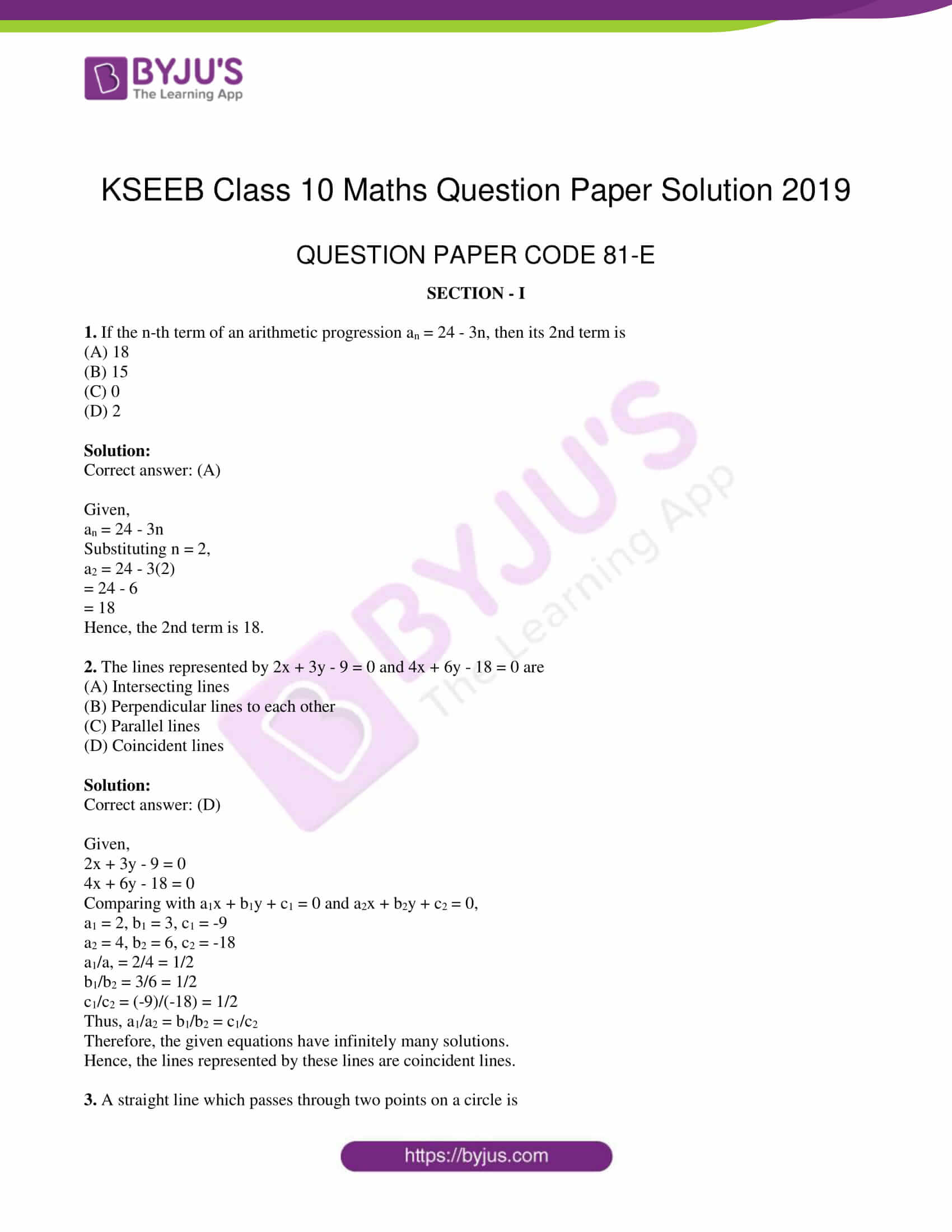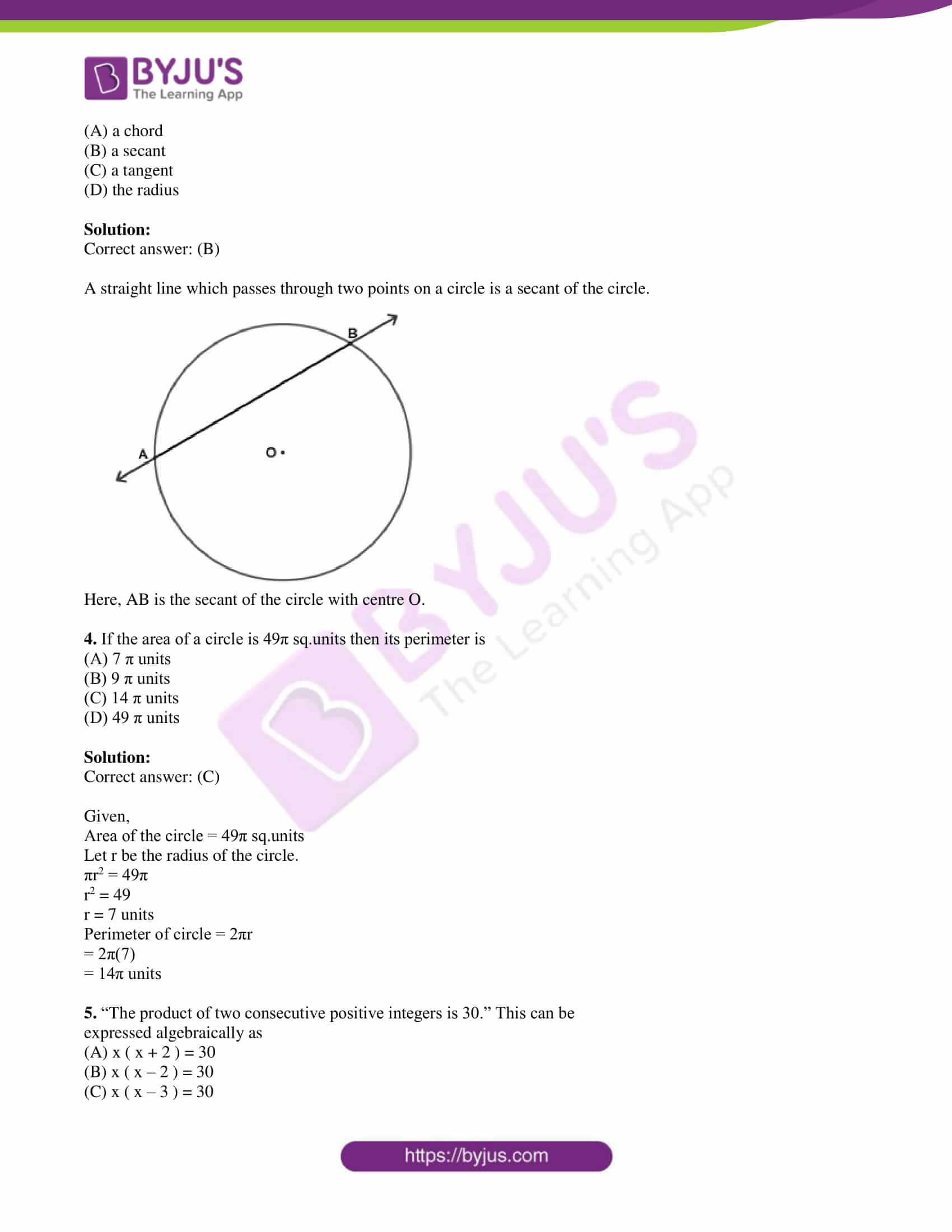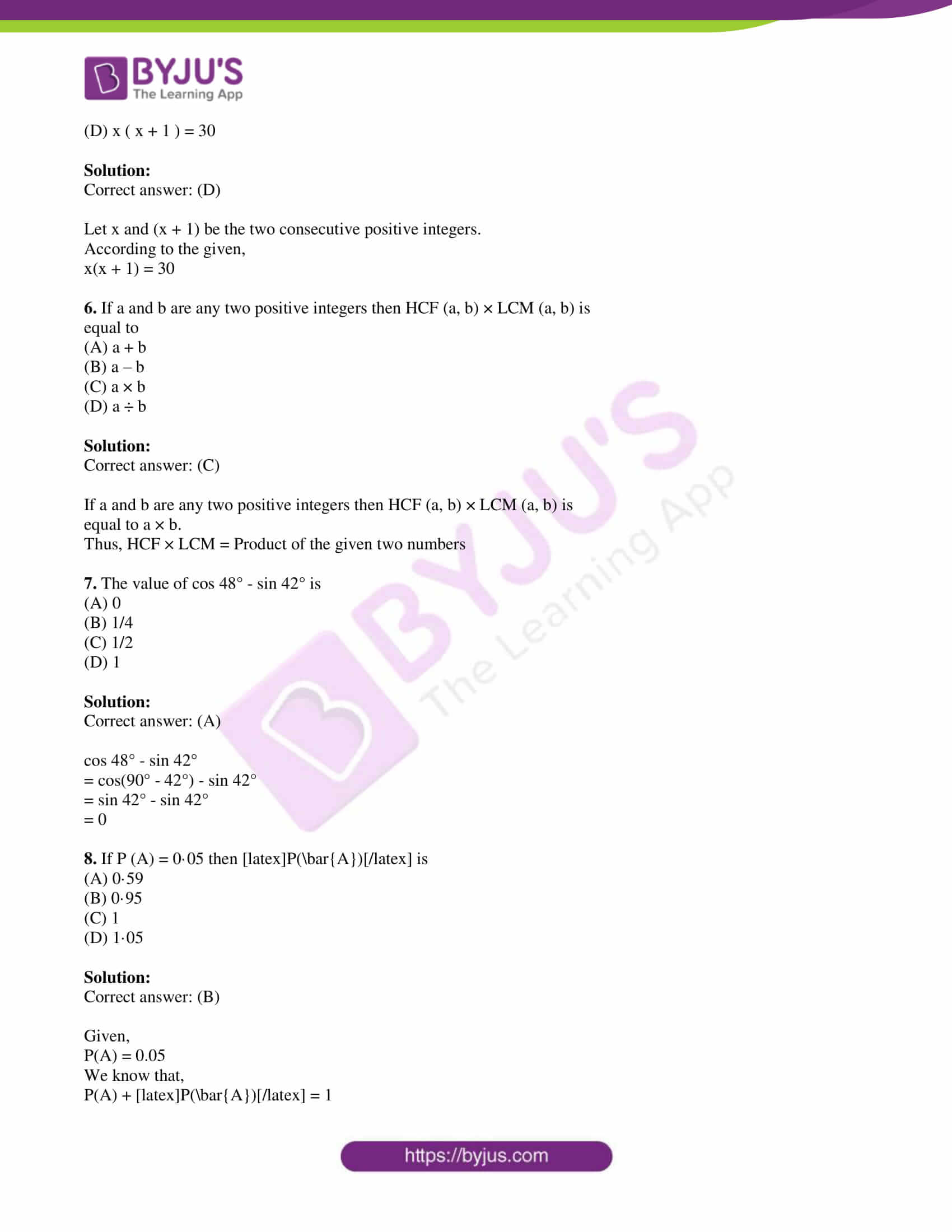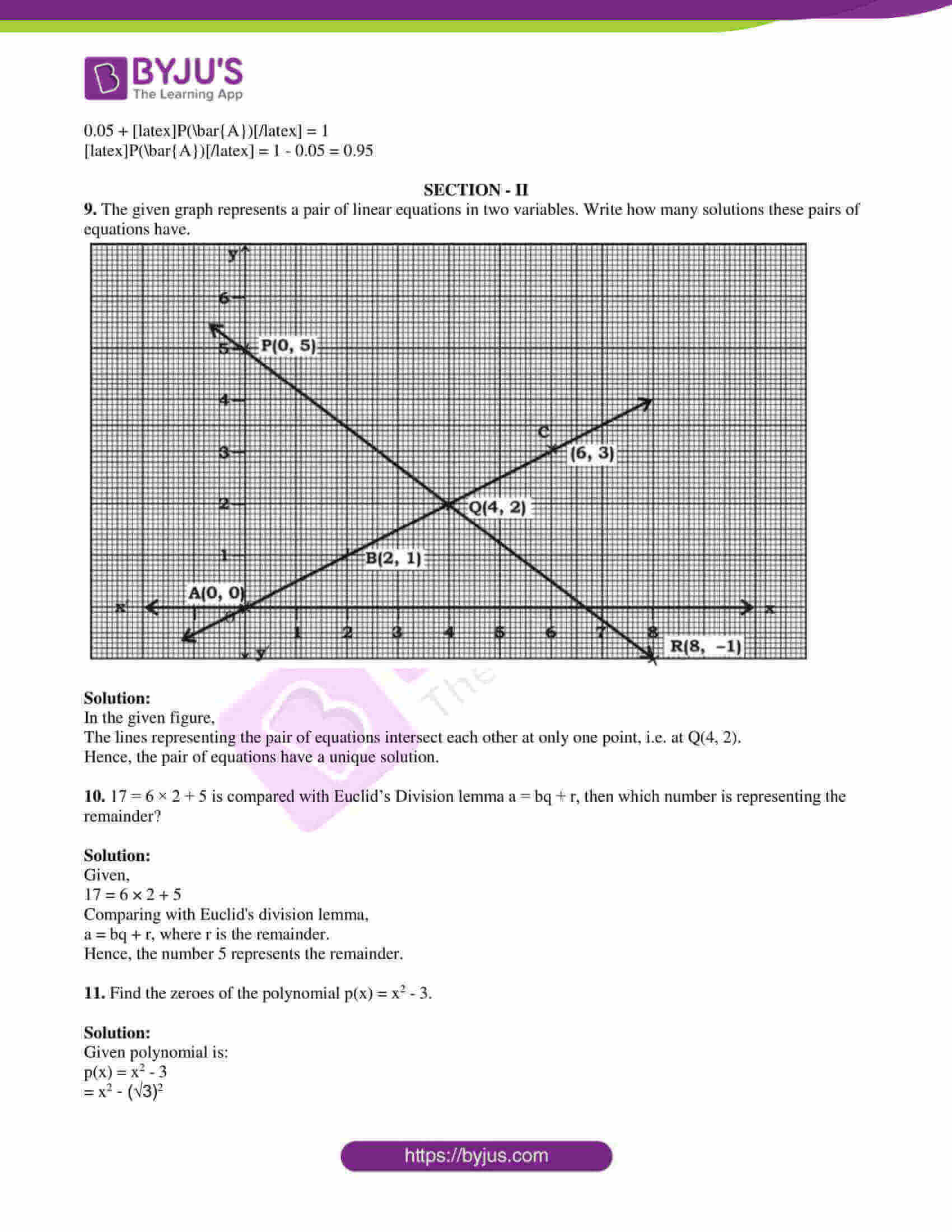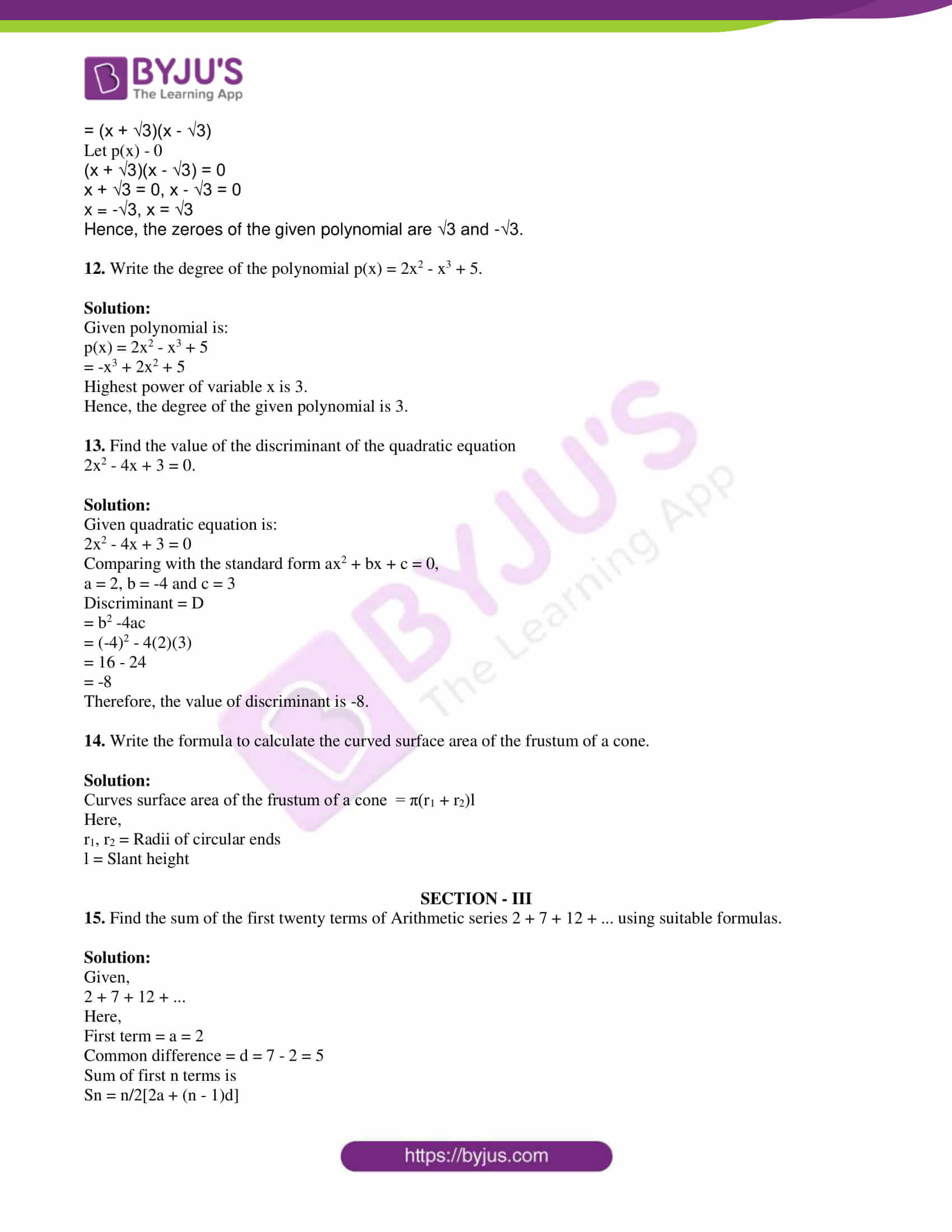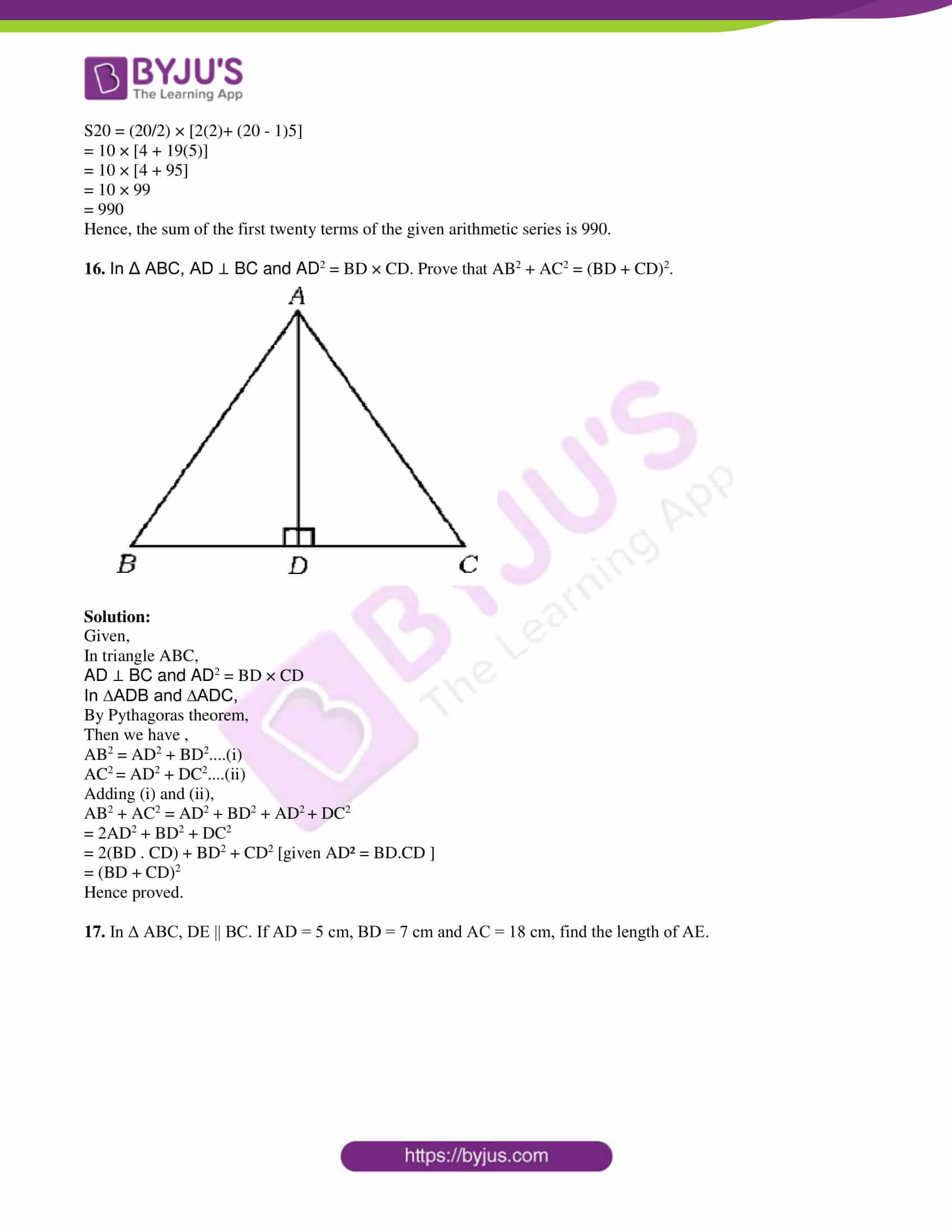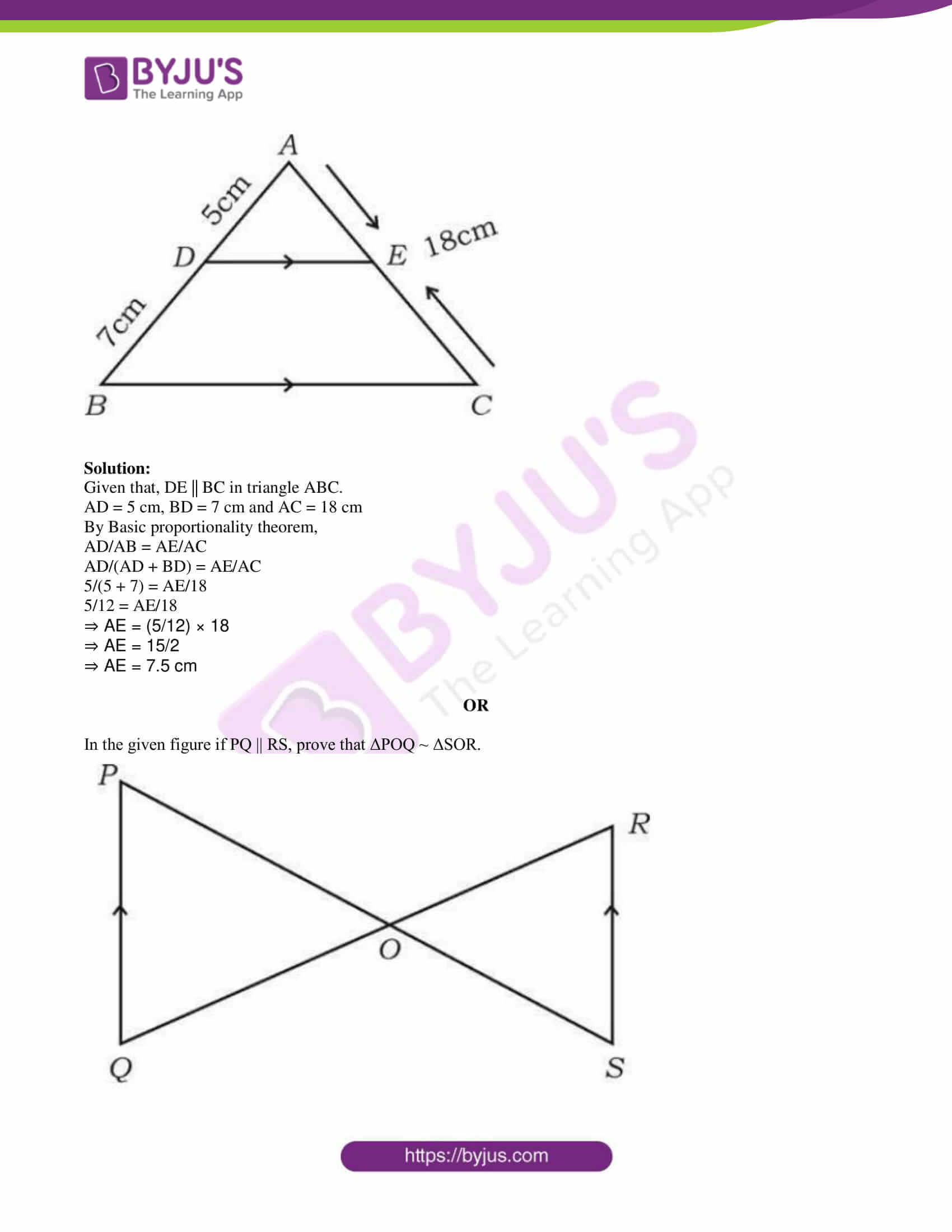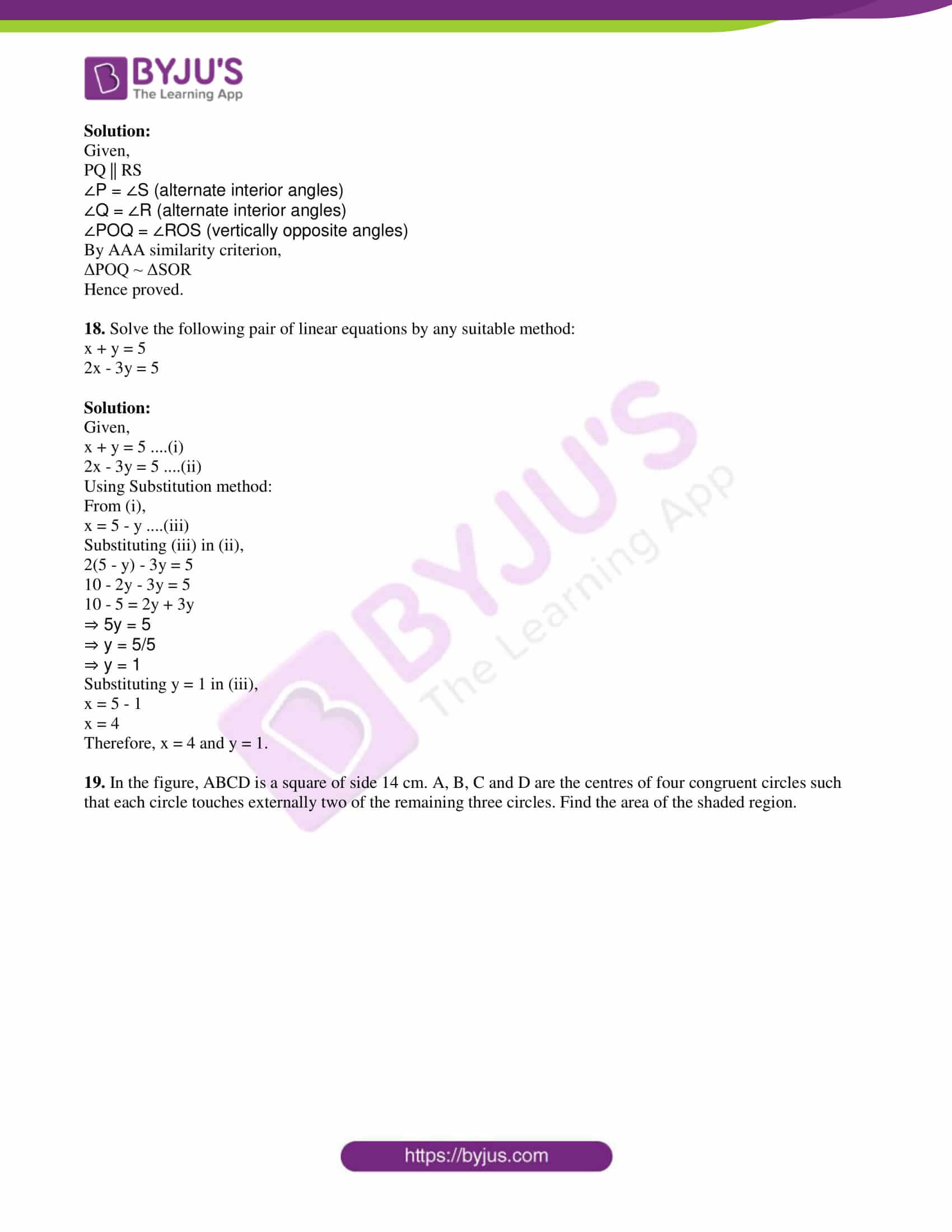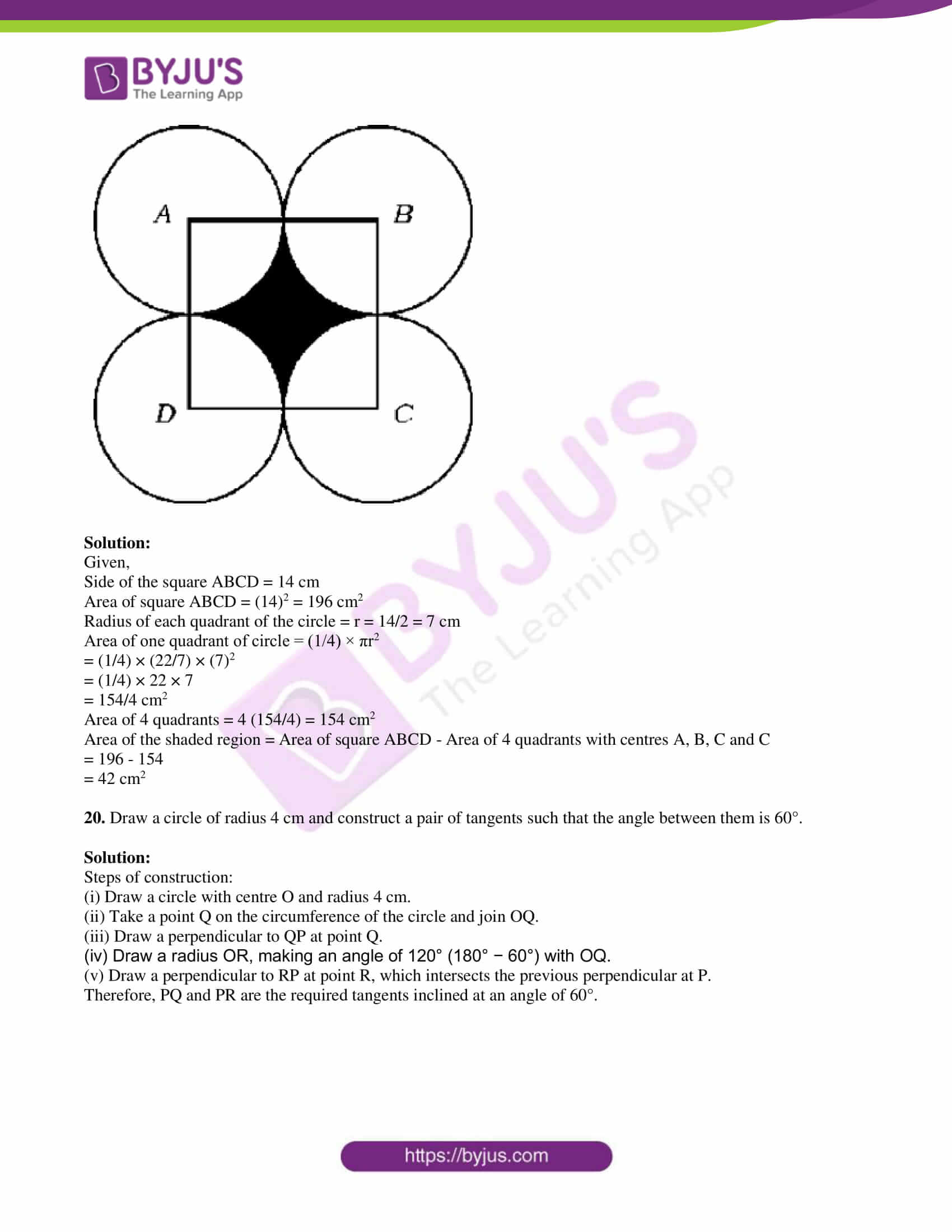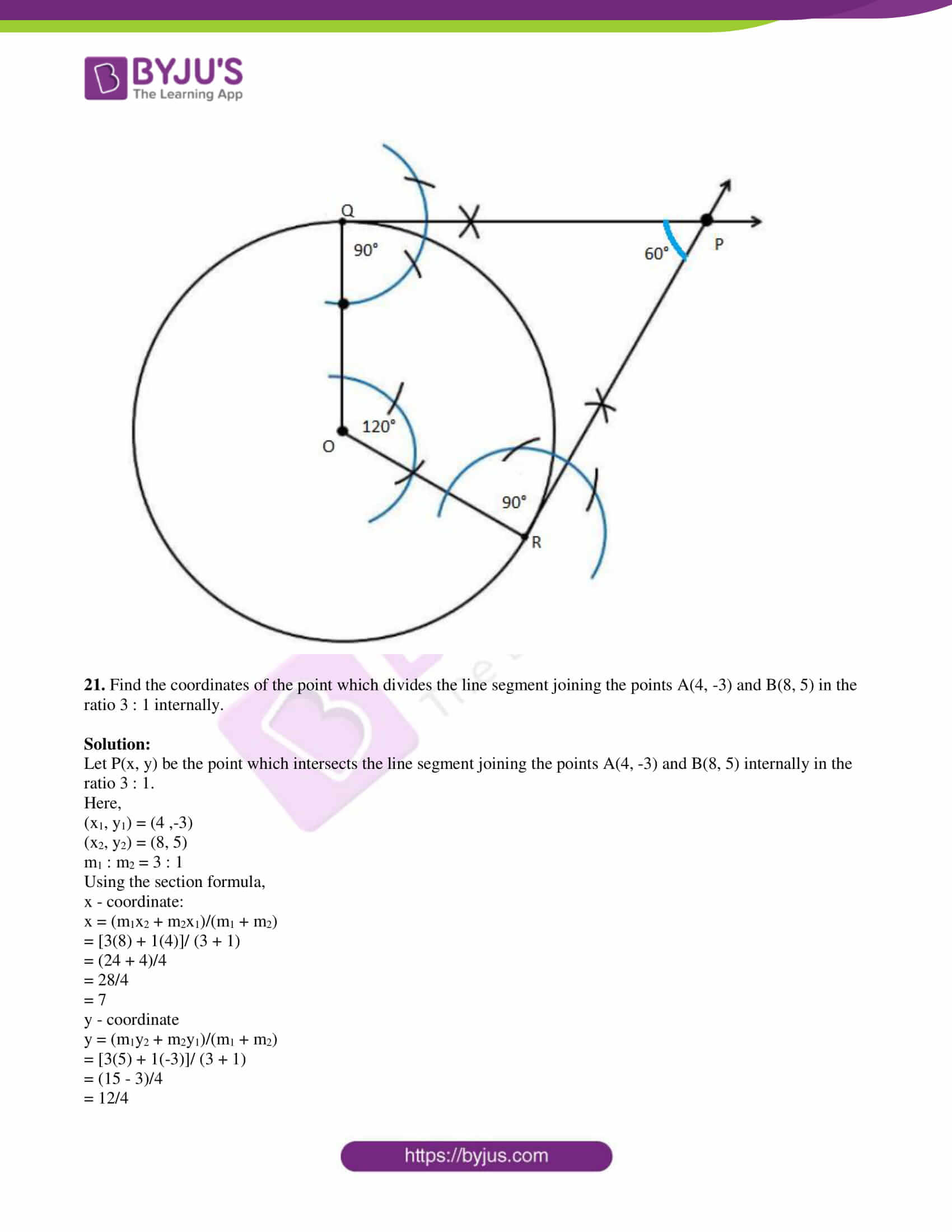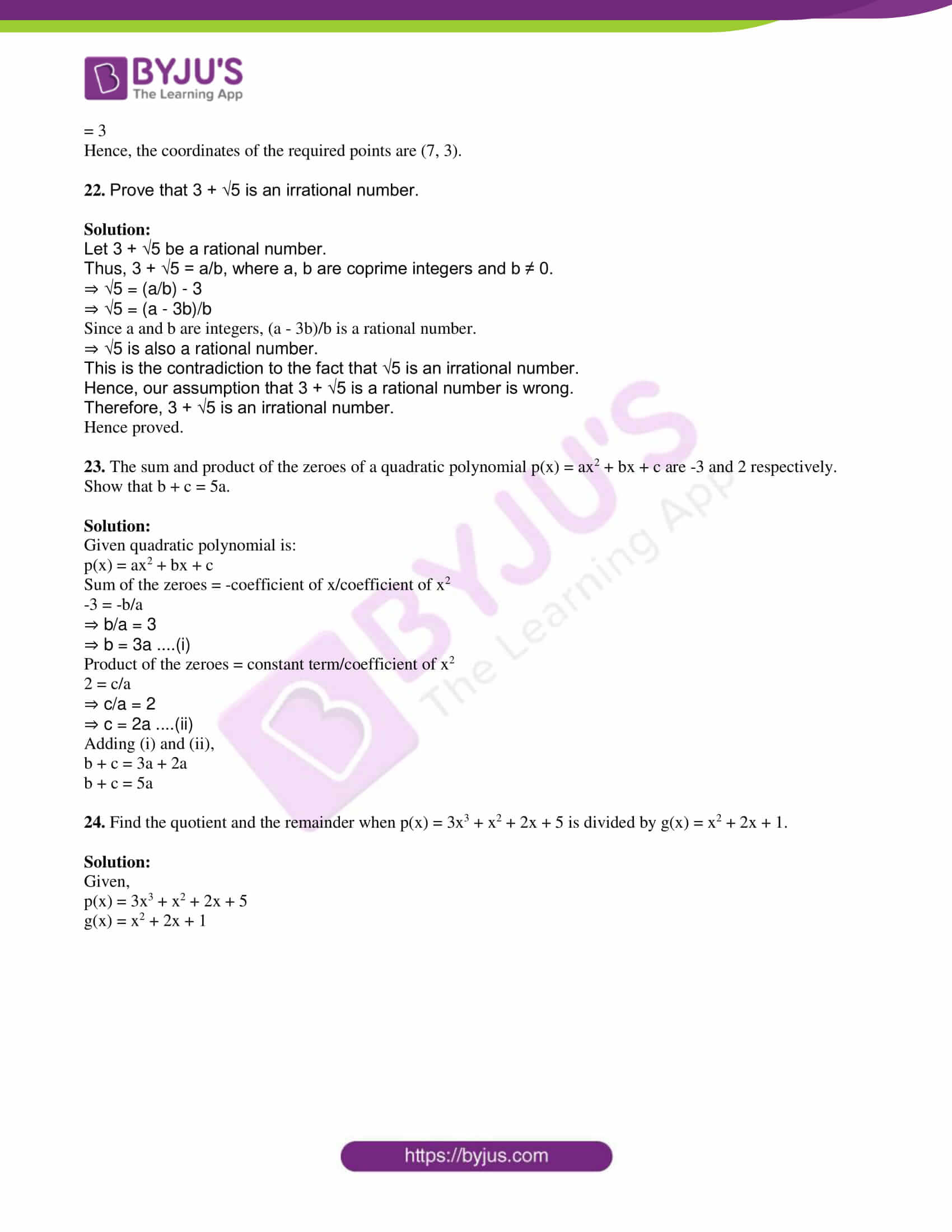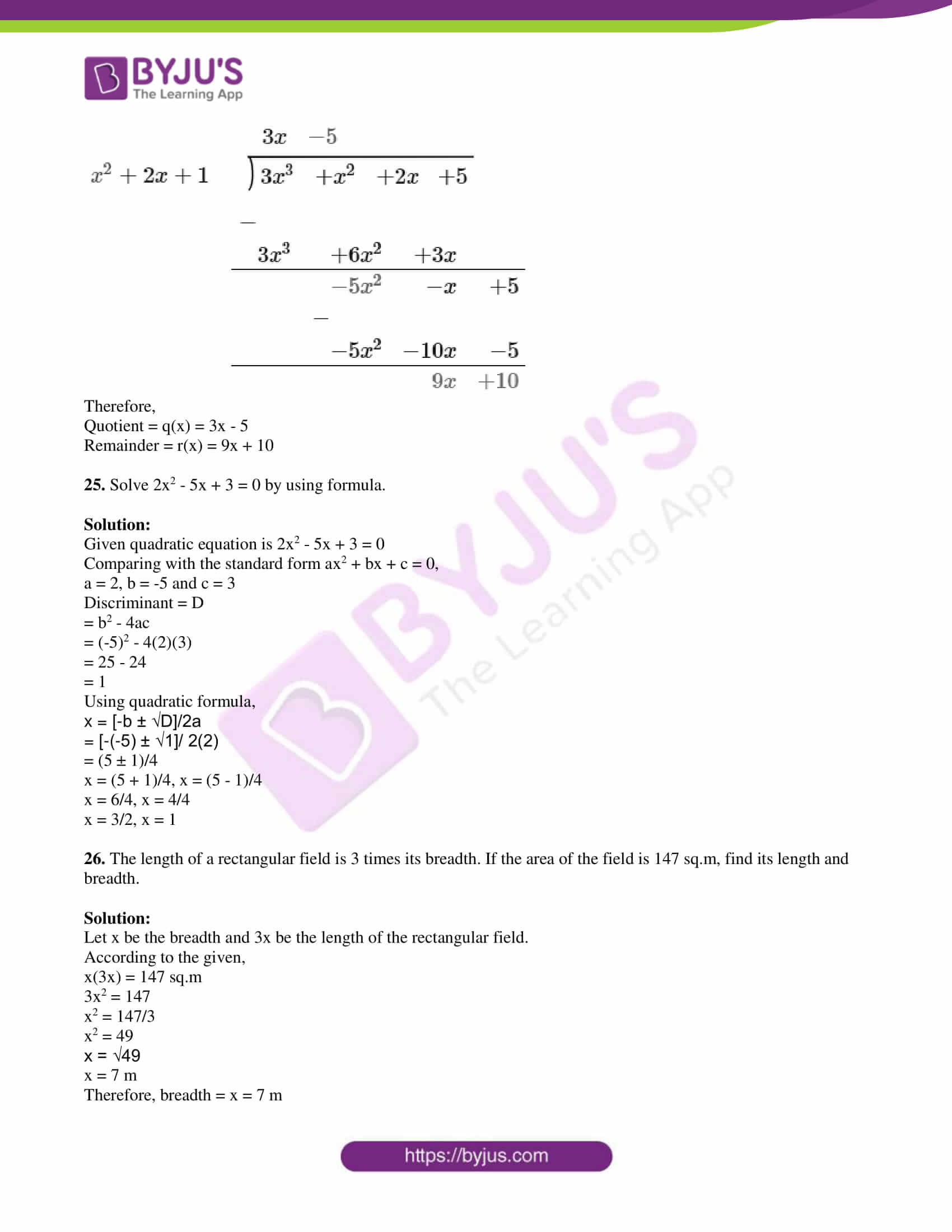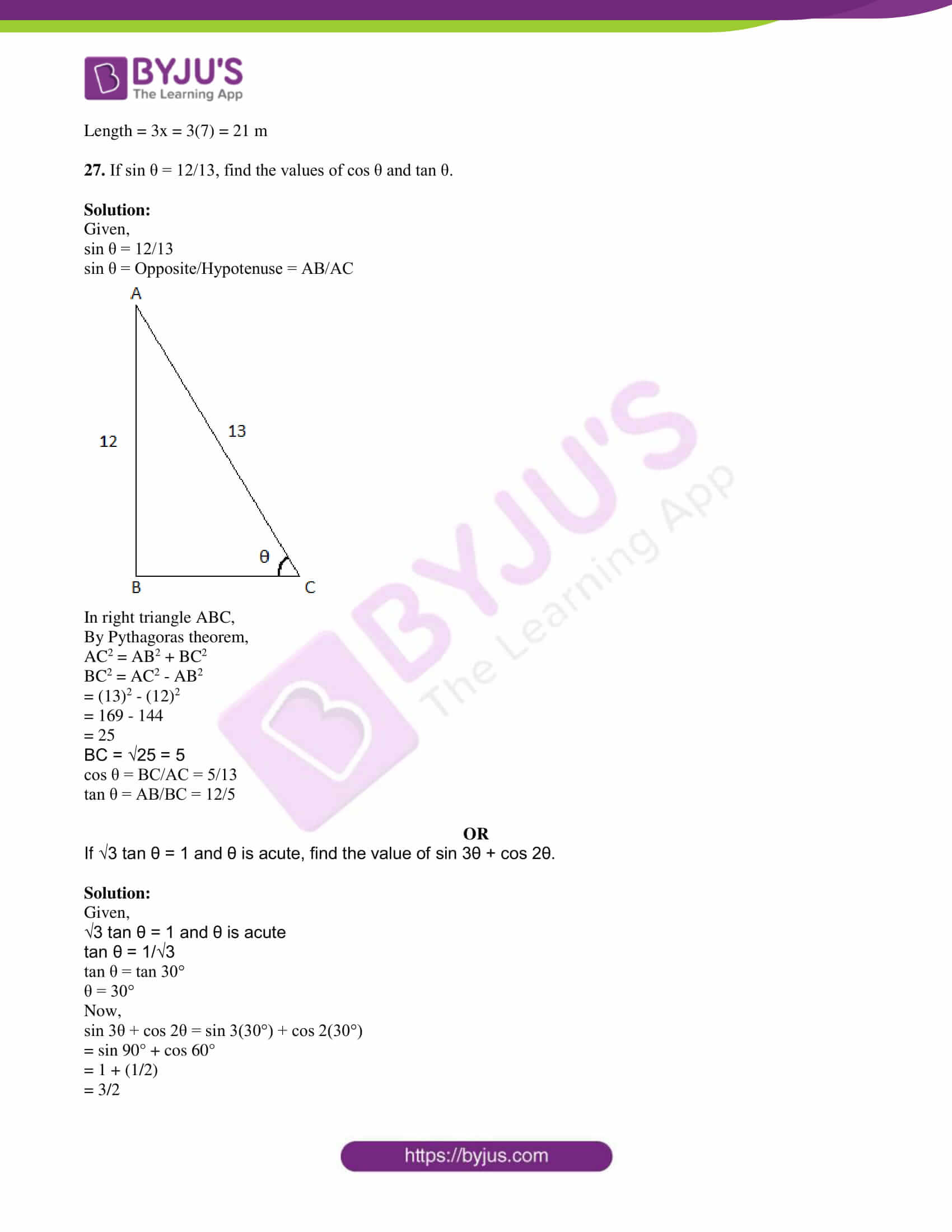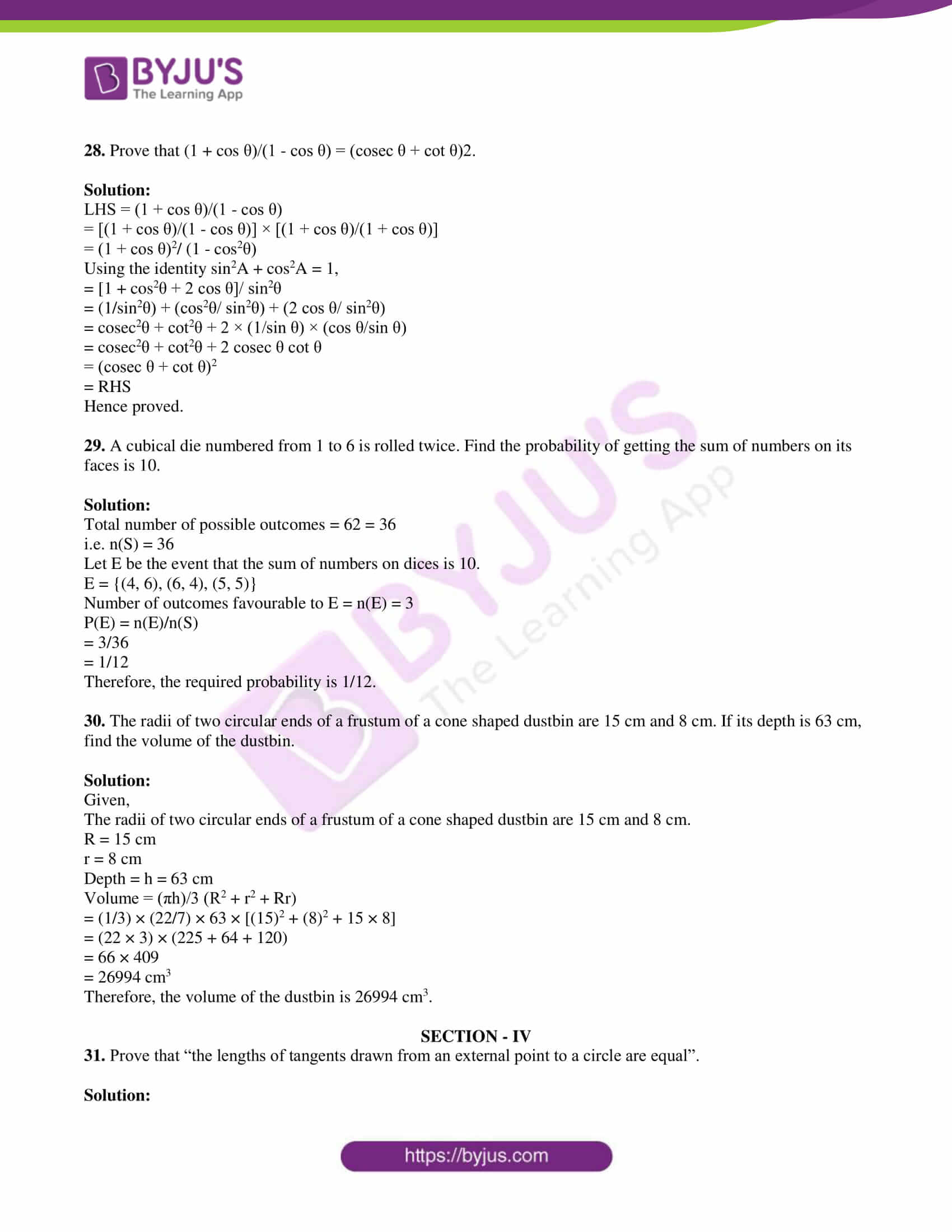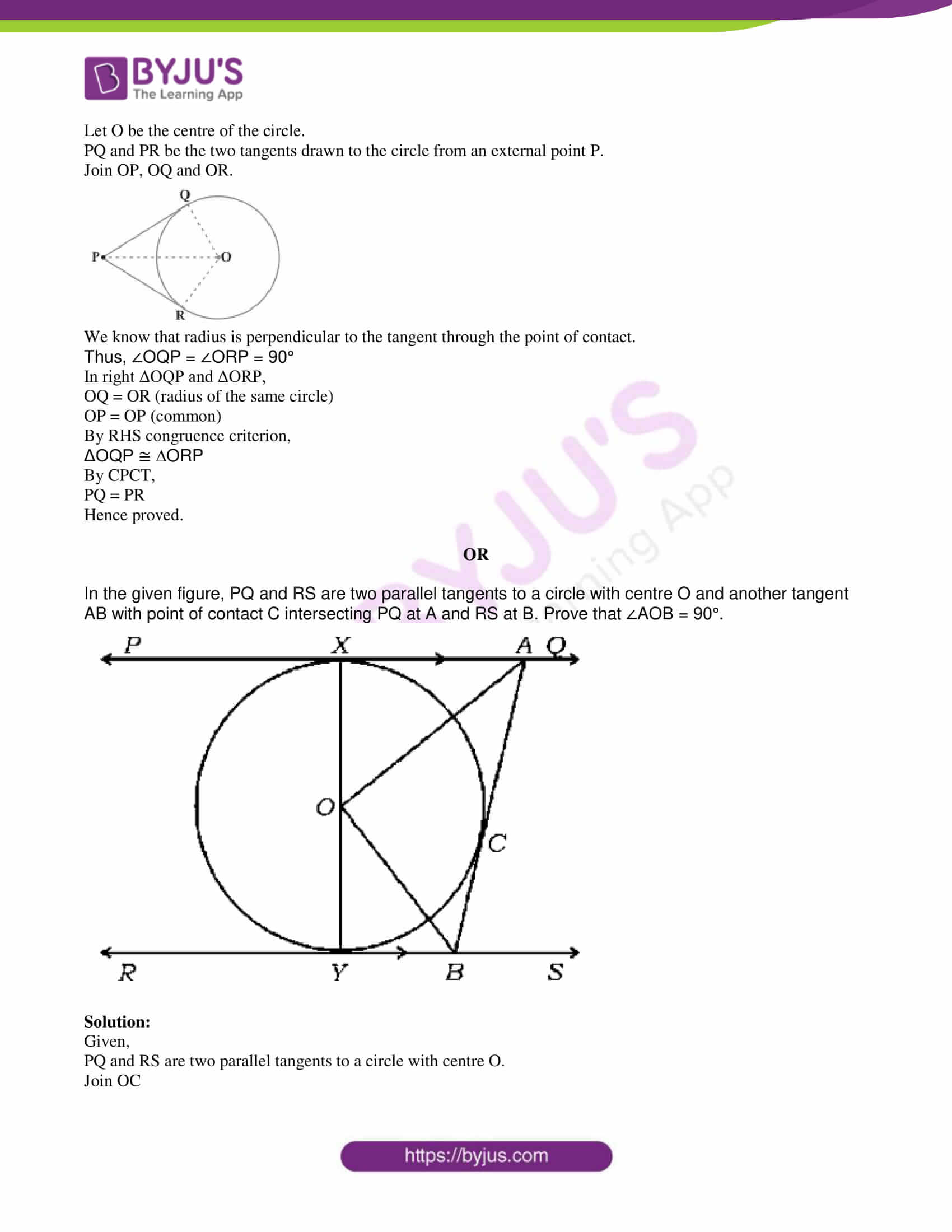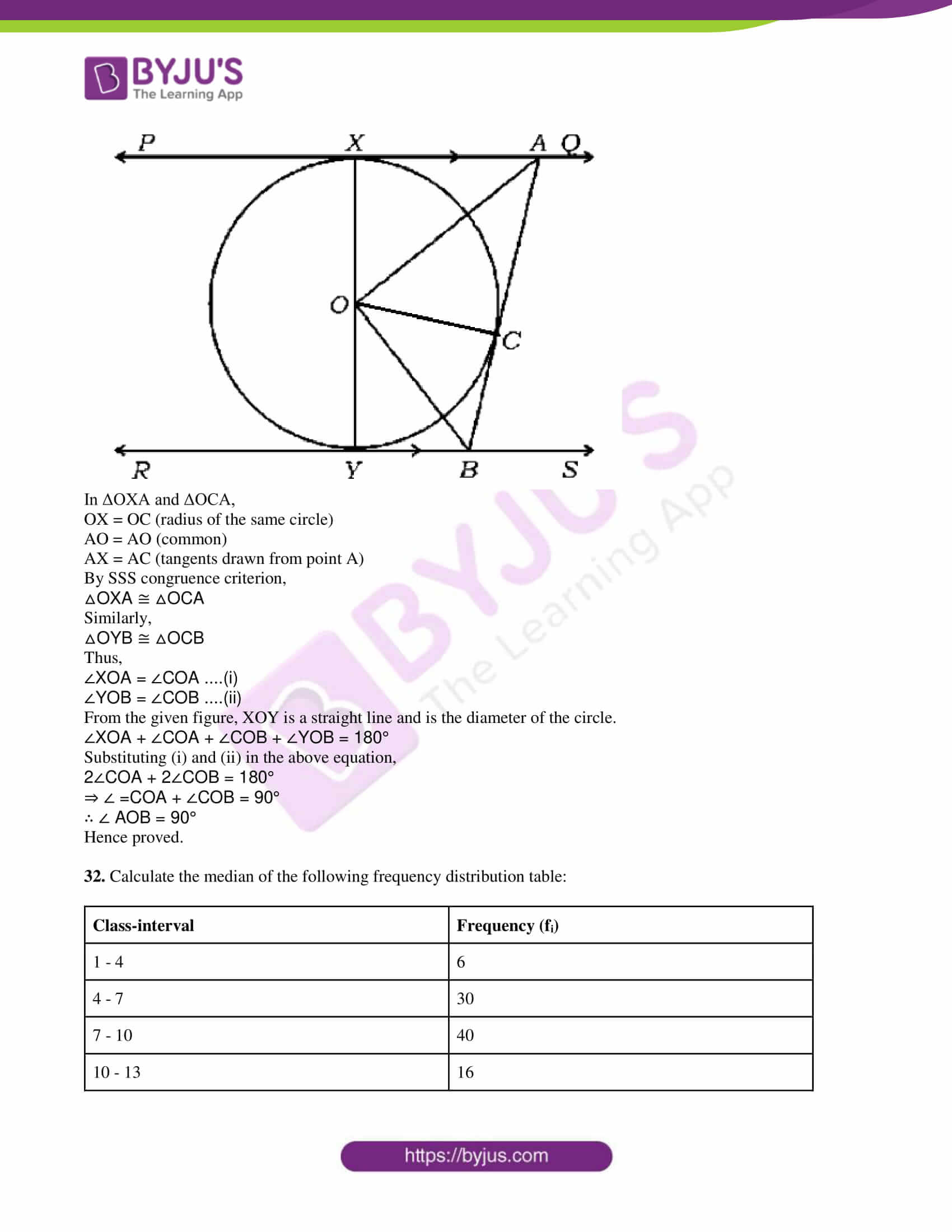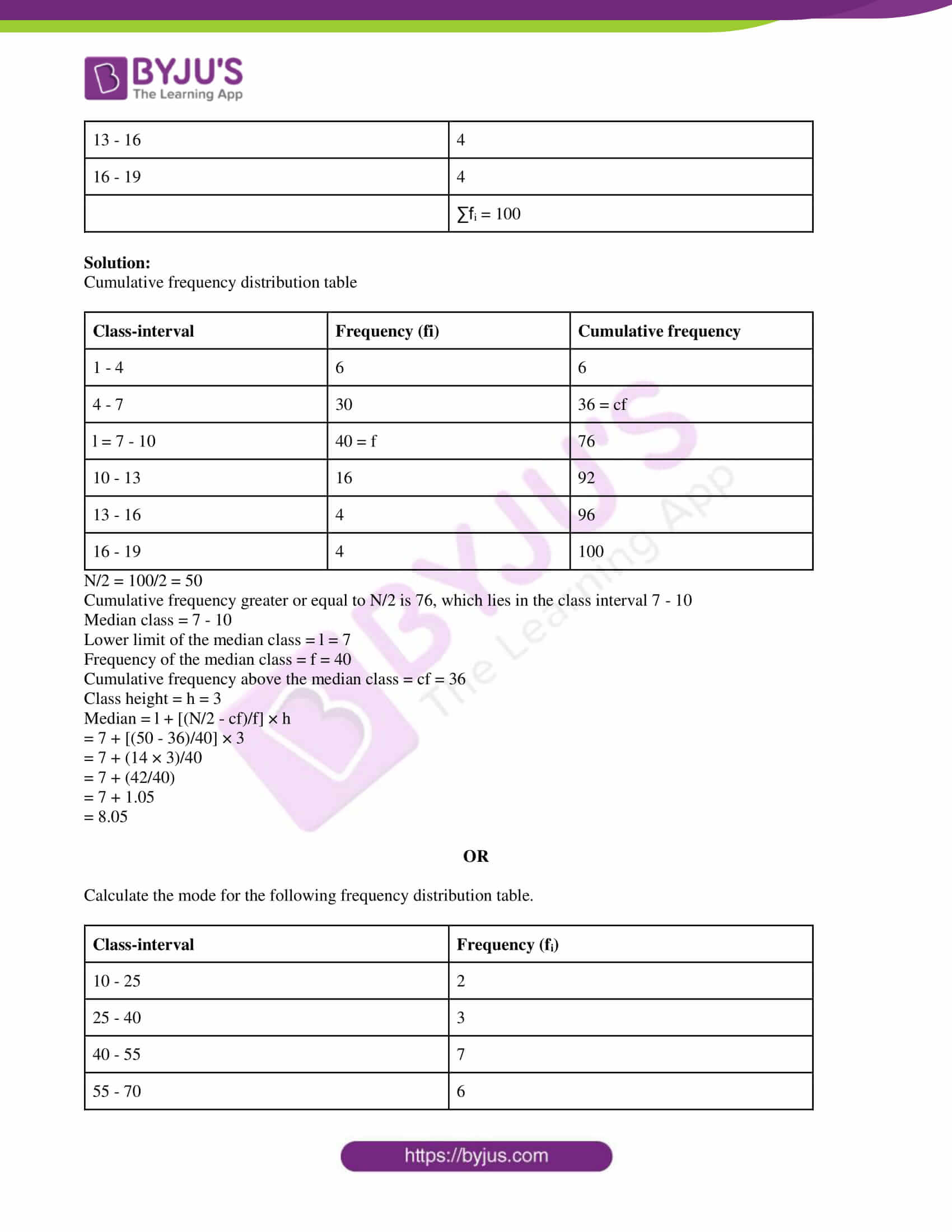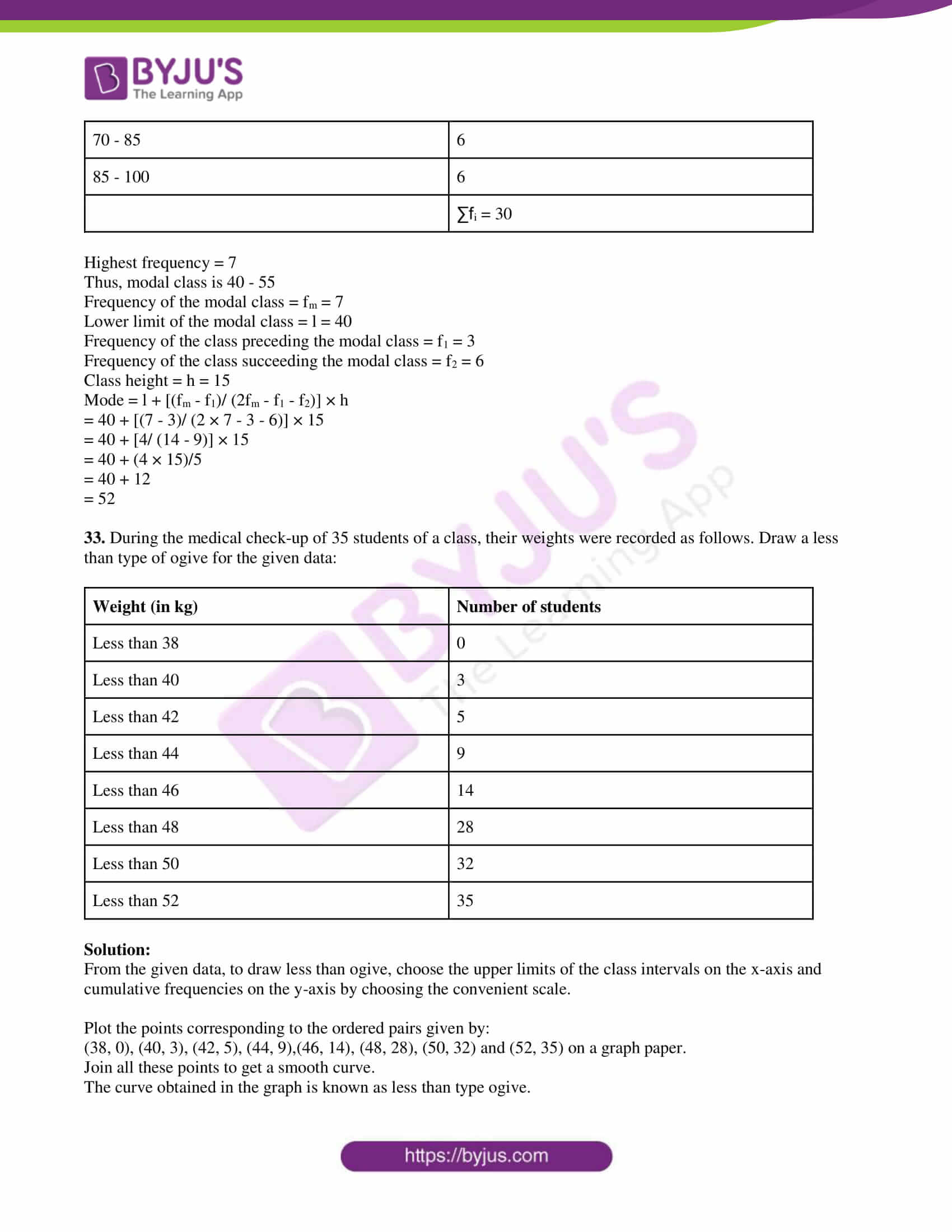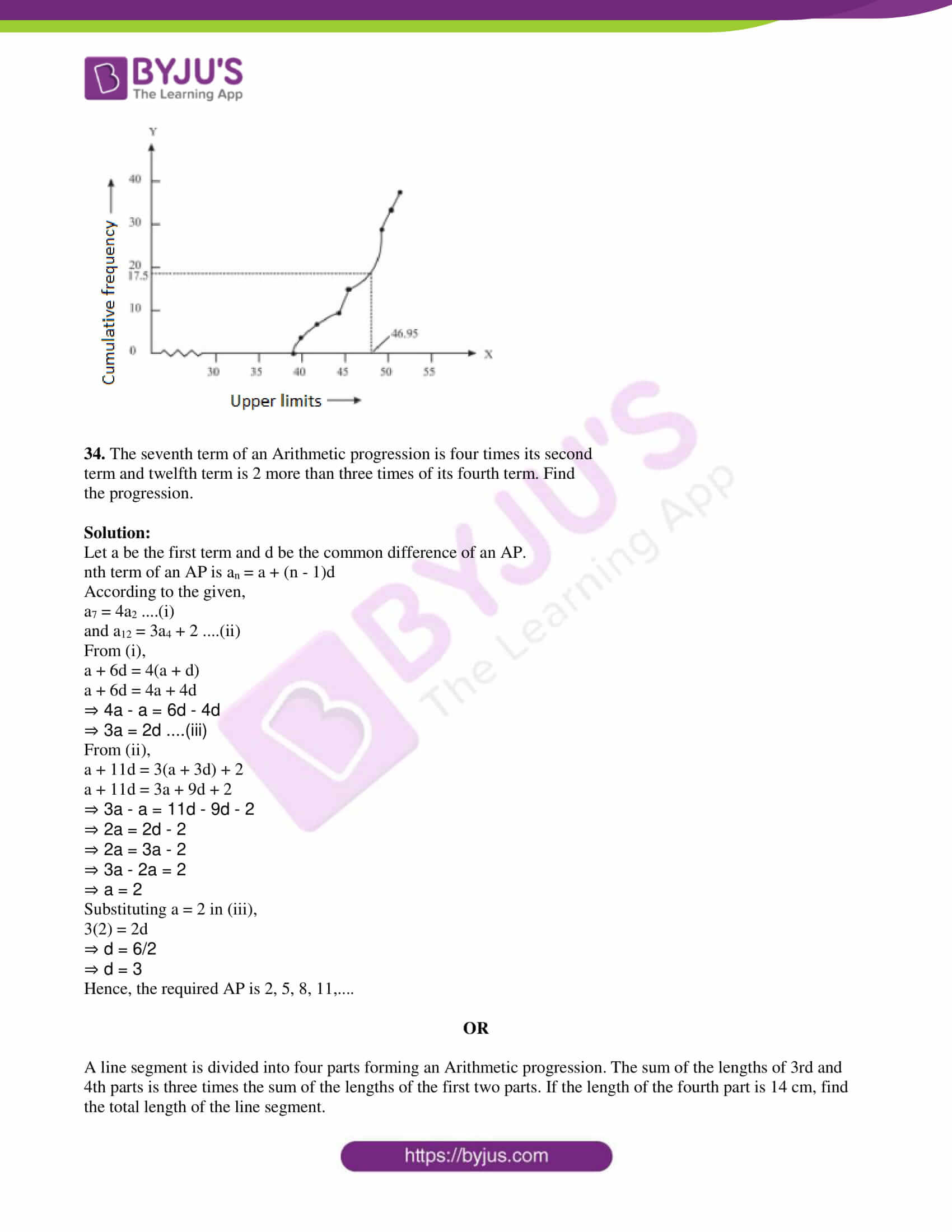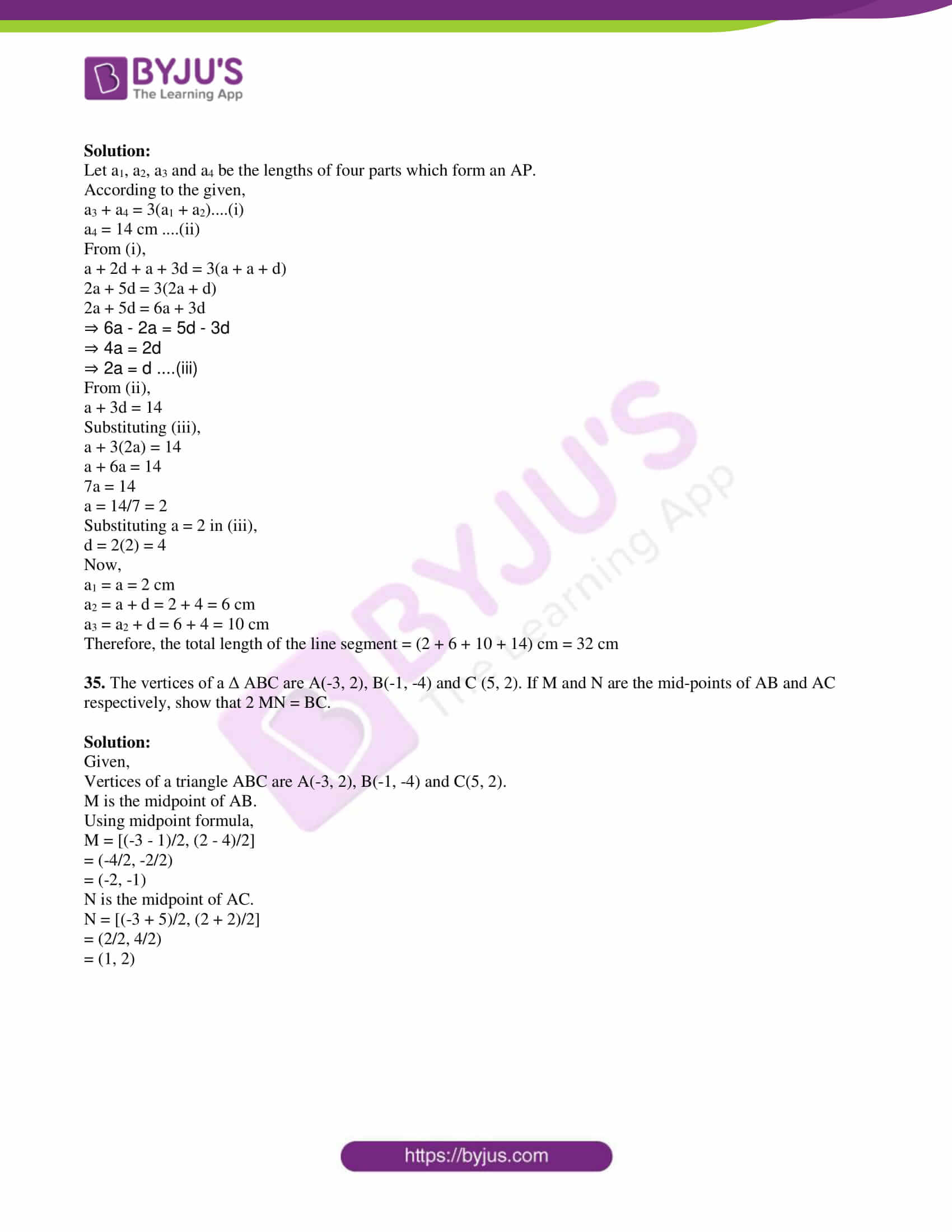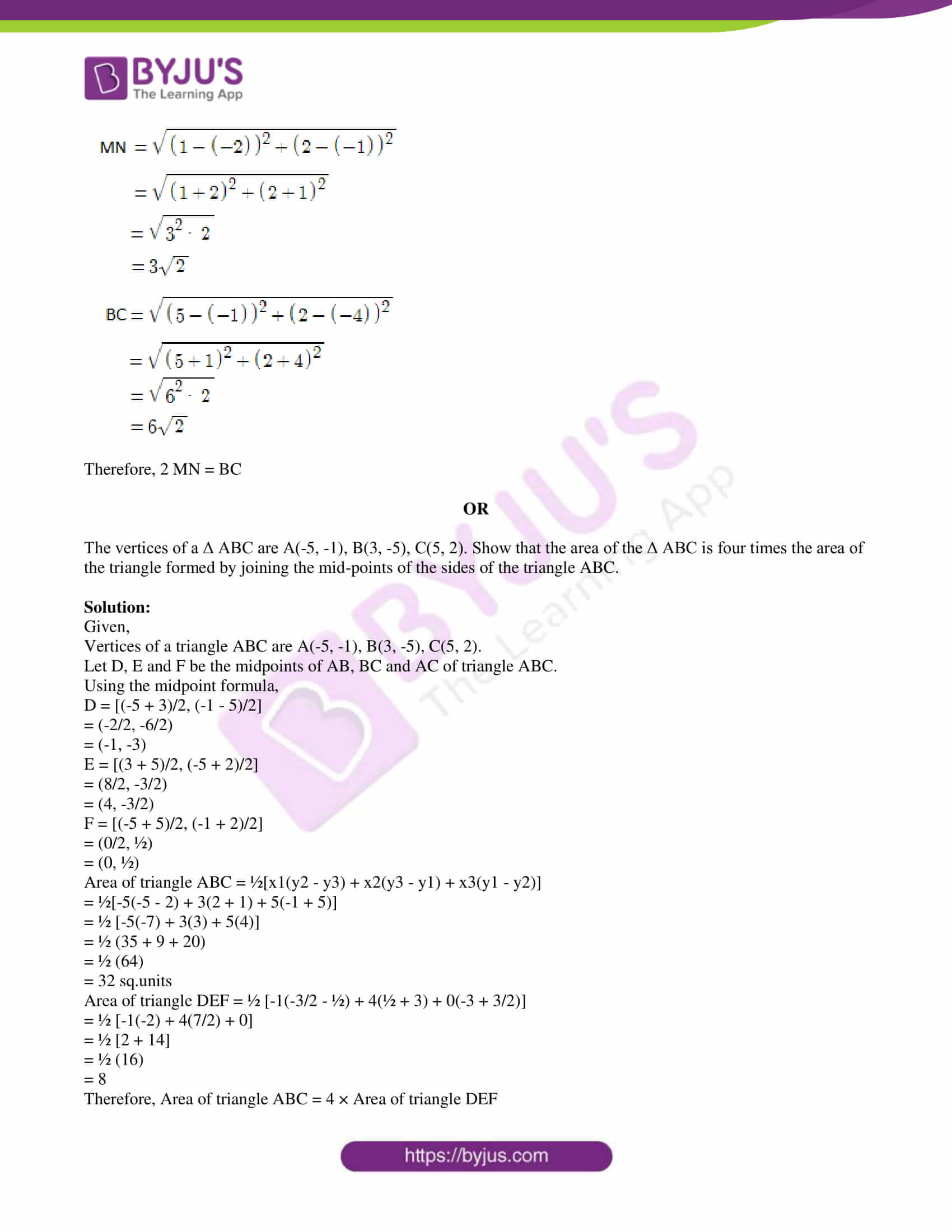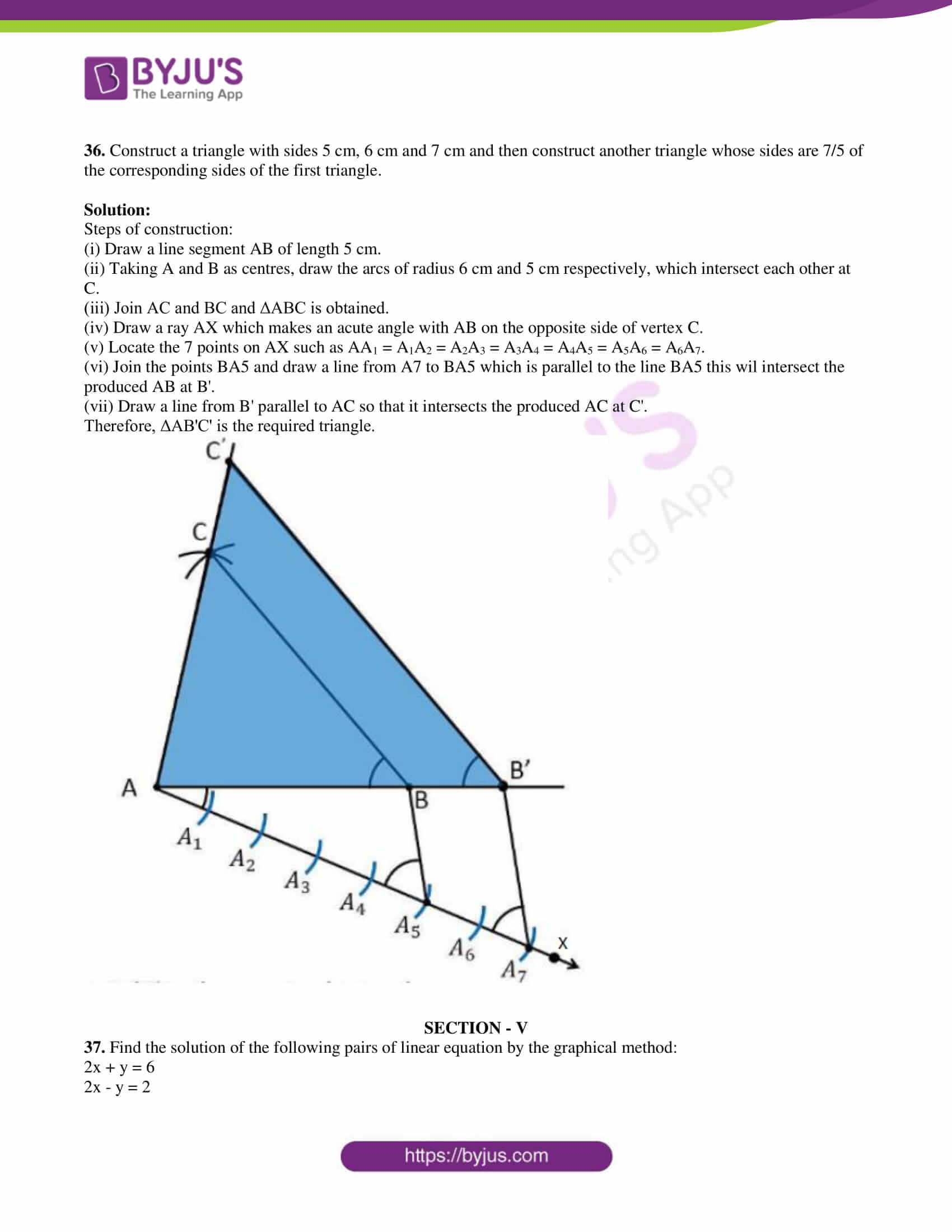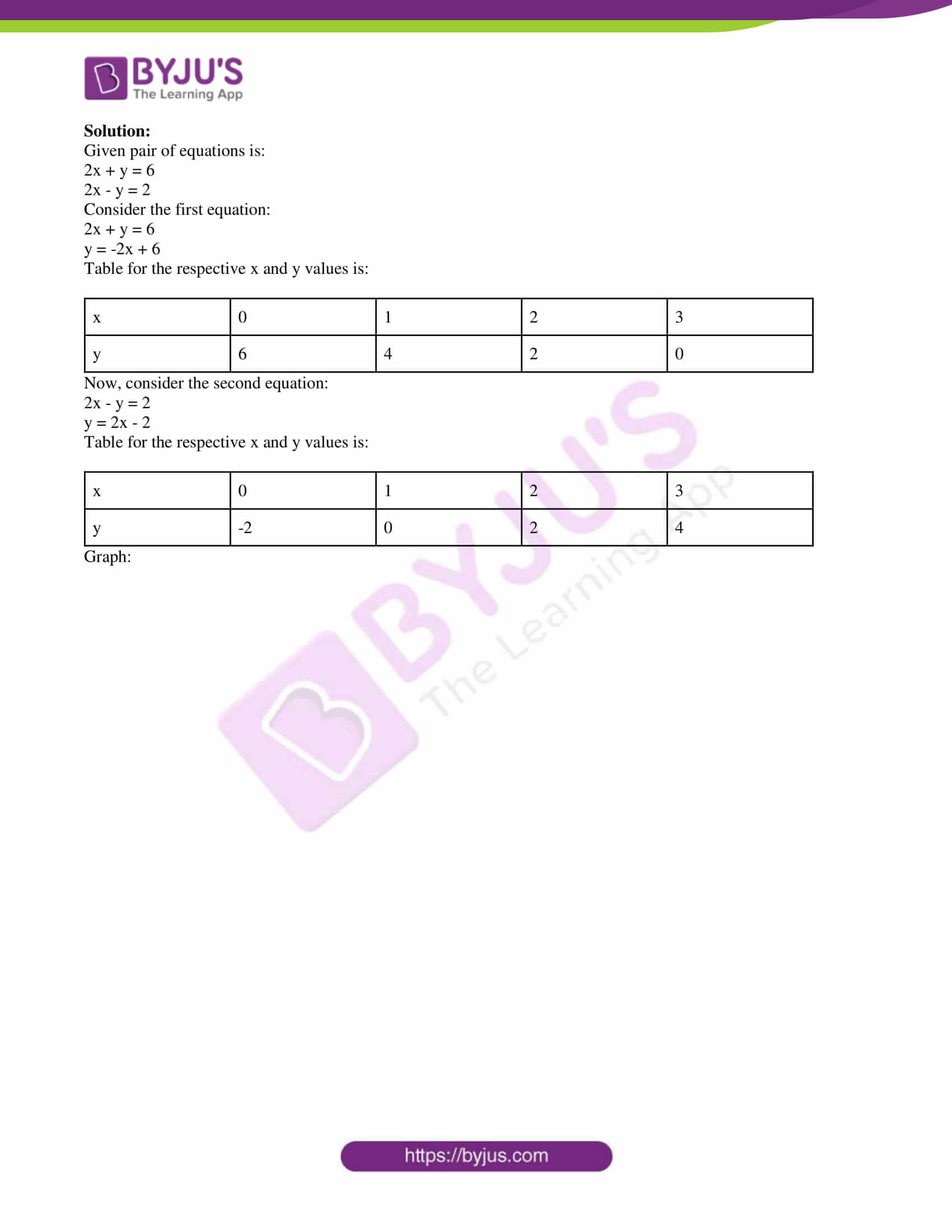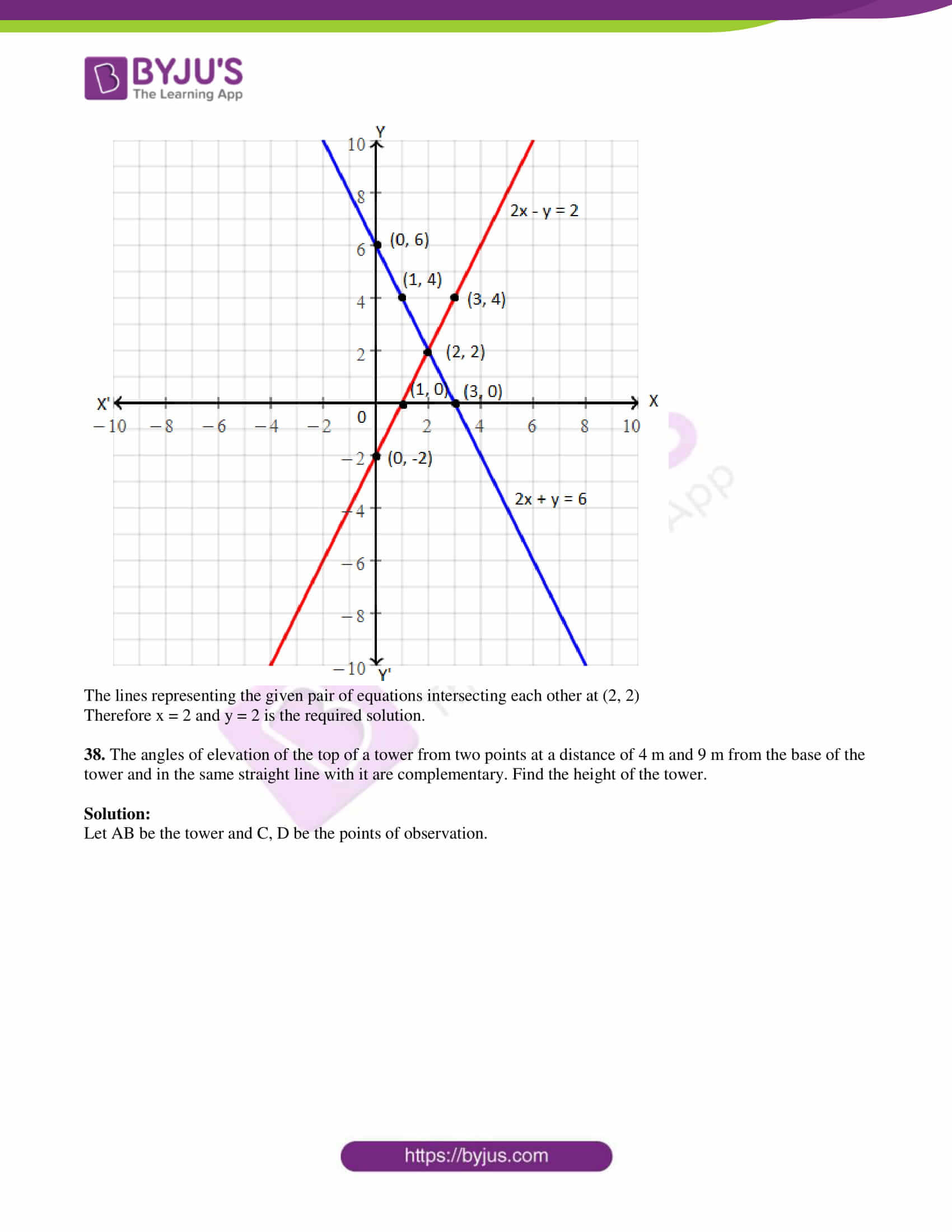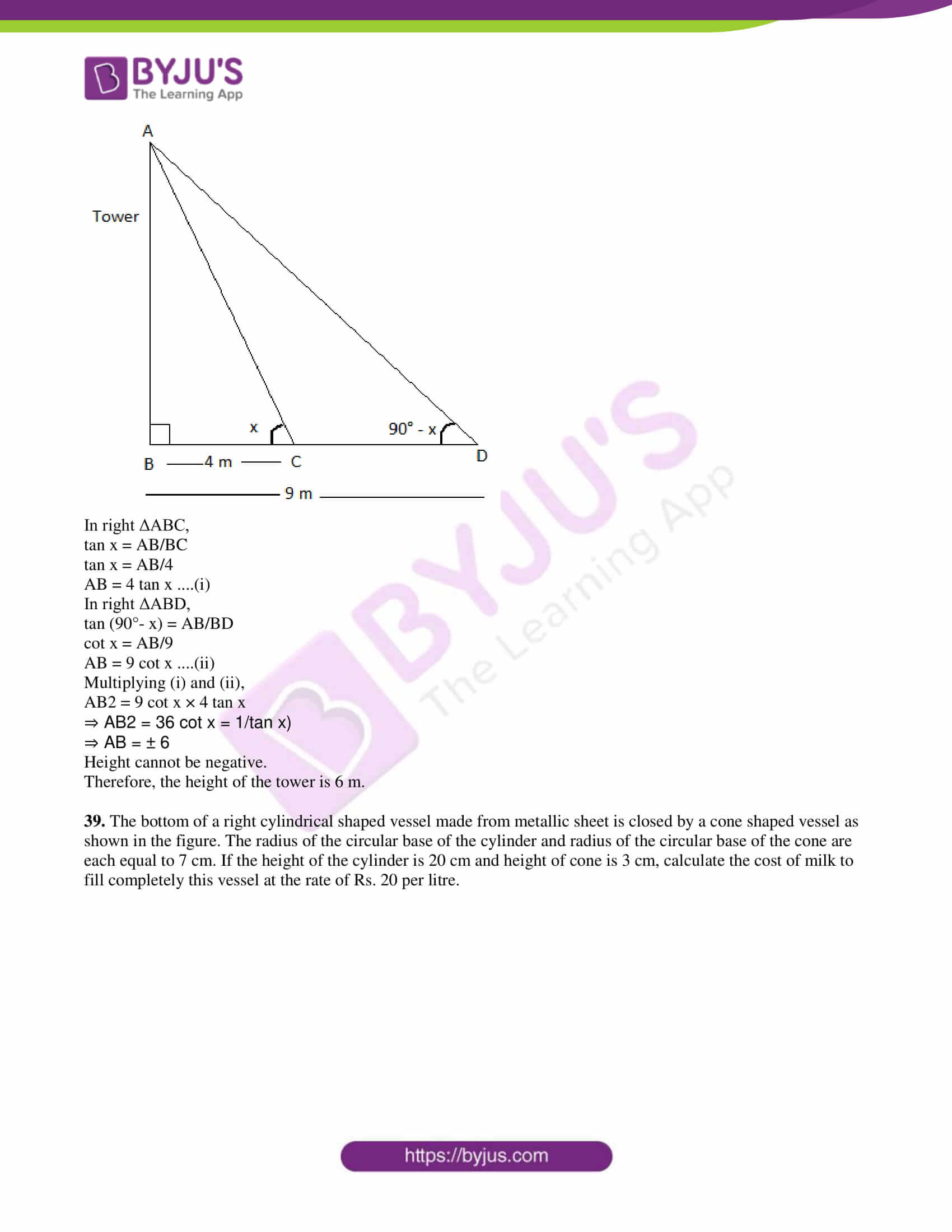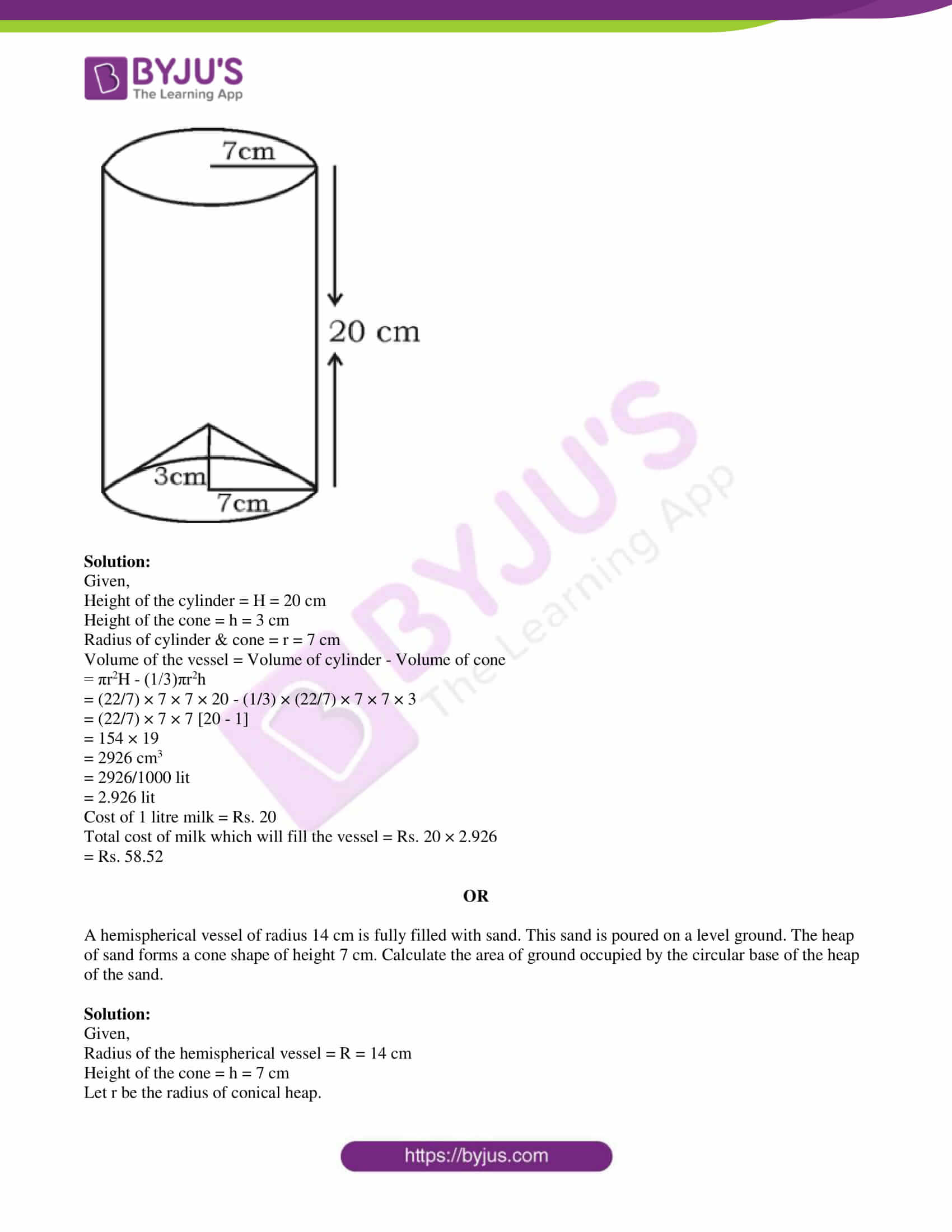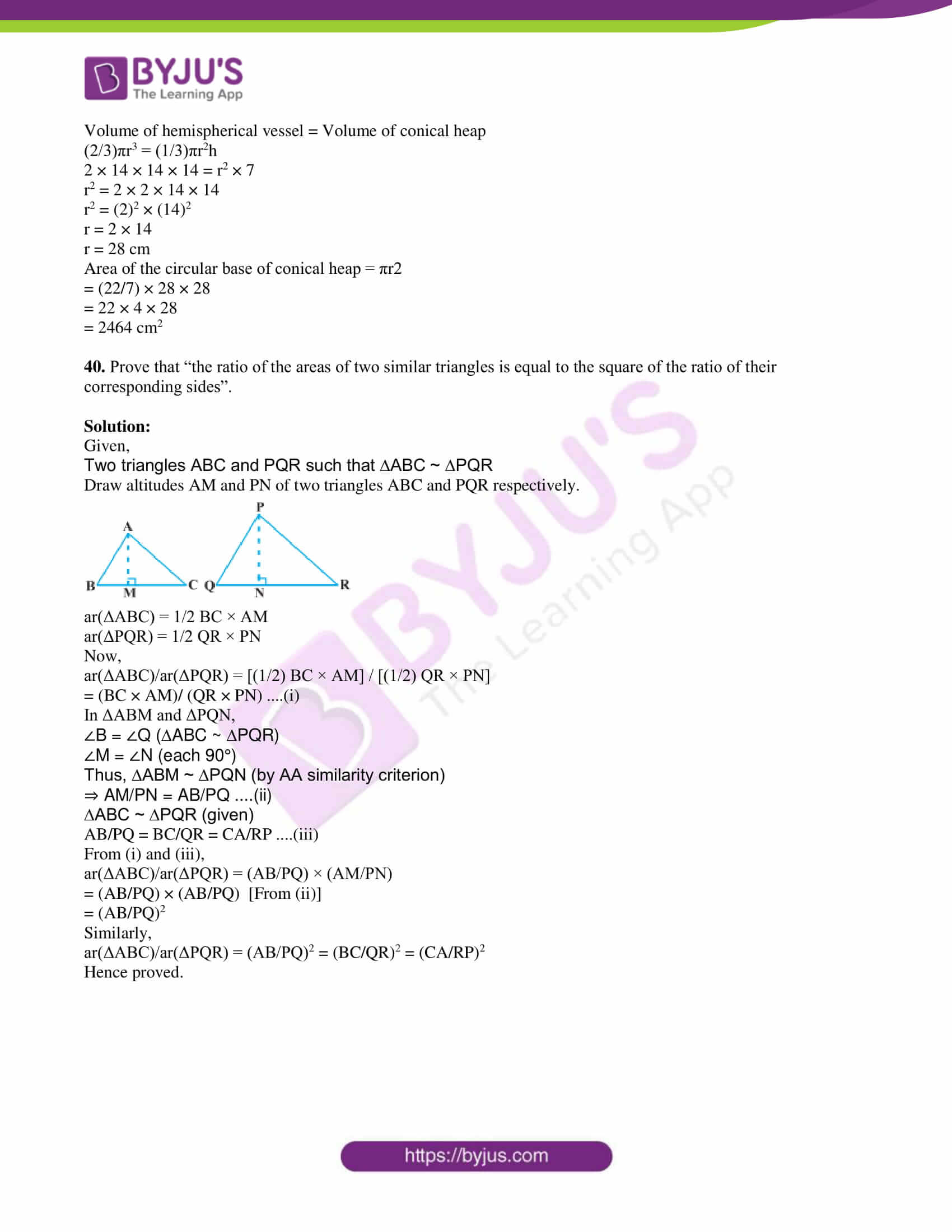### QUESTION PAPER CODE 81-E

SECTION – I

1. If the n-th term of an arithmetic progression an = 24 – 3n, then its 2nd term is

(A) 18

(B) 15

(C) 0

(D) 2

Solution:

Given,

an = 24 – 3n

Substituting n = 2,

a2 = 24 – 3(2)

= 24 – 6

= 18

Hence, the 2nd term is 18.

2. The lines represented by 2x + 3y – 9 = 0 and 4x + 6y – 18 = 0 are

(A) Intersecting lines

(B) Perpendicular lines to each other

(C) Parallel lines

(D) Coincident lines

Solution:

Given,

2x + 3y – 9 = 0

4x + 6y – 18 = 0

Comparing with a1x + b1y + c1 = 0 and a2x + b2y + c2 = 0,

a1 = 2, b1 = 3, c1 = -9

a2 = 4, b2 = 6, c2 = -18

a1/a, = 2/4 = 1/2

b1/b2 = 3/6 = 1/2

c1/c2 = (-9)/(-18) = 1/2

Thus, a1/a2 = b1/b2 = c1/c2

Therefore, the given equations have infinitely many solutions.

Hence, the lines represented by these lines are coincident lines.

3. A straight line which passes through two points on a circle is

(A) a chord

(B) a secant

(C) a tangent

Solution:

A straight line which passes through two points on a circle is a secant of the circle.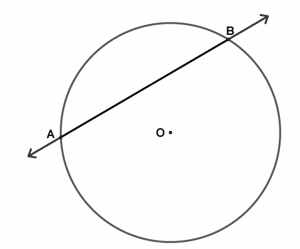Here, AB is the secant of the circle with centre O.

4. If the area of a circle is 49π sq.units then its perimeter is

(A) 7 π units

(B) 9 π units

(C) 14 π units

(D) 49 π units

Solution:

Given,

Area of the circle = 49π sq.units

Let r be the radius of the circle.

πr2 = 49π

r2 = 49

r = 7 units

Perimeter of circle = 2πr

= 2π(7)

= 14π units

5. “The product of two consecutive positive integers is 30.” This can be

expressed algebraically as

(A) x ( x + 2 ) = 30

(B) x ( x – 2 ) = 30

(C) x ( x – 3 ) = 30

(D) x ( x + 1 ) = 30

Solution:

Let x and (x + 1) be the two consecutive positive integers.

According to the given,

x(x + 1) = 30

6. If a and b are any two positive integers then HCF (a, b) × LCM (a, b) is

equal to

(A) a + b

(B) a – b

(C) a × b

(D) a ÷ b

Solution:

If a and b are any two positive integers then HCF (a, b) × LCM (a, b) is

equal to a × b.

Thus, HCF × LCM = Product of the given two numbers

7. The value of cos 48° – sin 42° is

(A) 0

(B) 1/4

(C) 1/2

(D) 1

Solution:

cos 48° – sin 42°

= cos(90° – 42°) – sin 42°

= sin 42° – sin 42°

= 0

8. If P (A) = 0·05 then

$$\begin{array}{l}P(\bar{A})\end{array}$$
is

(A) 0·59

(B) 0·95

(C) 1

(D) 1·05

Solution:

Given,

P(A) = 0.05

We know that,

P(A) +

$$\begin{array}{l}P(\bar{A})\end{array}$$
= 1

0.05 +

$$\begin{array}{l}P(\bar{A})\end{array}$$
= 1

$$\begin{array}{l}P(\bar{A})\end{array}$$
= 1 – 0.05 = 0.95

SECTION – II

9. The given graph represents a pair of linear equations in two variables. Write how many solutions these pairs of equations have.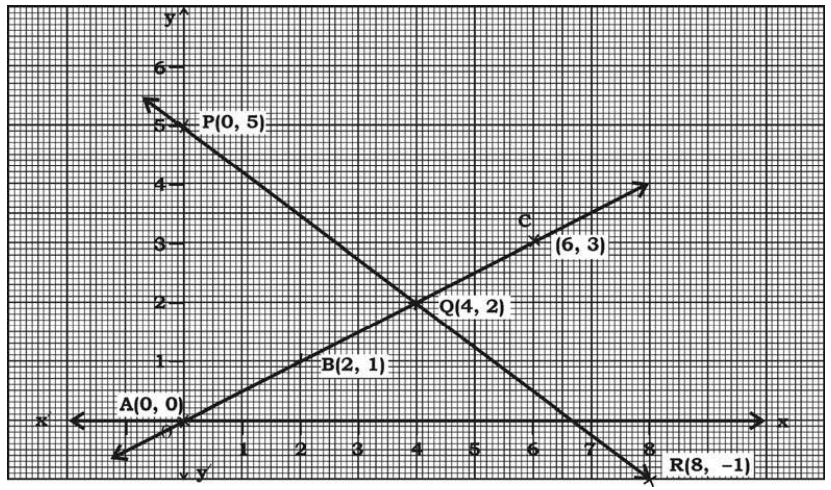Solution:

In the given figure,

The lines representing the pair of equations intersect each other at only one point, i.e. at Q(4, 2).

Hence, the pair of equations have a unique solution.

10. 17 = 6 × 2 + 5 is compared with Euclid’s Division lemma a = bq + r, then which number is representing the remainder?

Solution:

Given,

17 = 6 × 2 + 5

Comparing with Euclid’s division lemma,

a = bq + r, where r is the remainder.

Hence, the number 5 represents the remainder.

11. Find the zeroes of the polynomial p(x) = x2 – 3.

Solution:

Given polynomial is:

p(x) = x2 – 3

= x2 – (√3)2

= (x + √3)(x – √3)

Let p(x) – 0

(x + √3)(x – √3) = 0

x + √3 = 0, x – √3 = 0

x = -√3, x = √3

Hence, the zeroes of the given polynomial are √3 and -√3.

12. Write the degree of the polynomial p(x) = 2x2 – x3 + 5.

Solution:

Given polynomial is:

p(x) = 2x2 – x3 + 5

= -x3 + 2x2 + 5

Highest power of variable x is 3.

Hence, the degree of the given polynomial is 3.

13. Find the value of the discriminant of the quadratic equation

2x2 – 4x + 3 = 0.

Solution:

2x2 – 4x + 3 = 0

Comparing with the standard form ax2 + bx + c = 0,

a = 2, b = -4 and c = 3

Discriminant = D

= b2 -4ac

= (-4)2 – 4(2)(3)

= 16 – 24

= -8

Therefore, the value of discriminant is -8.

14. Write the formula to calculate the curved surface area of the frustum of a cone.

Solution:

Curves surface area of the frustum of a cone = π(r1 + r2)l

Here,

r1, r2 = Radii of circular ends

l = Slant height

SECTION – III

15. Find the sum of the first twenty terms of Arithmetic series 2 + 7 + 12 + … using suitable formulas.

Solution:

Given,

2 + 7 + 12 + …

Here,

First term = a = 2

Common difference = d = 7 – 2 = 5

Sum of first n terms is

Sn = n/2[2a + (n – 1)d]

S20 = (20/2) × [2(2)+ (20 – 1)5]

= 10 × [4 + 19(5)]

= 10 × [4 + 95]

= 10 × 99

= 990

Hence, the sum of the first twenty terms of the given arithmetic series is 990.

16. In Δ ABC, AD ⊥ BC and AD2 = BD × CD. Prove that AB2 + AC2 = (BD + CD)2.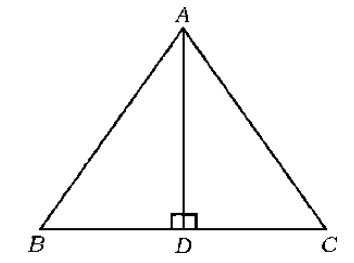Solution:

Given,

In triangle ABC,

By Pythagoras theorem,

Then we have ,

= 2AD2 + BD2 + DC2

= 2(BD . CD) + BD2 + CD2 [given AD² = BD.CD ]

= (BD + CD)2

Hence proved.

17. In Δ ABC, DE || BC. If AD = 5 cm, BD = 7 cm and AC = 18 cm, find the length of AE.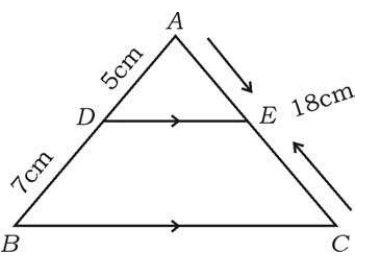Solution:

Given that, DE || BC in triangle ABC.

AD = 5 cm, BD = 7 cm and AC = 18 cm

By Basic proportionality theorem,

5/(5 + 7) = AE/18

5/12 = AE/18

⇒ AE = (5/12) × 18

⇒ AE = 15/2

⇒ AE = 7.5 cm

OR

In the given figure if PQ || RS, prove that ΔPOQ ~ ΔSOR.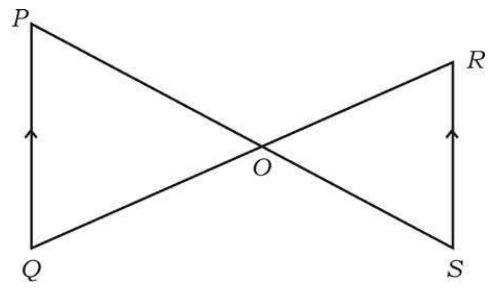Solution:

Given,

PQ || RS

∠P = ∠S (alternate interior angles)

∠Q = ∠R (alternate interior angles)

∠POQ = ∠ROS (vertically opposite angles)

By AAA similarity criterion,

ΔPOQ ~ ΔSOR

Hence proved.

18. Solve the following pair of linear equations by any suitable method:

x + y = 5

2x – 3y = 5

Solution:

Given,

x + y = 5 ….(i)

2x – 3y = 5 ….(ii)

Using Substitution method:

From (i),

x = 5 – y ….(iii)

Substituting (iii) in (ii),

2(5 – y) – 3y = 5

10 – 2y – 3y = 5

10 – 5 = 2y + 3y

⇒ 5y = 5

⇒ y = 5/5

⇒ y = 1

Substituting y = 1 in (iii),

x = 5 – 1

x = 4

Therefore, x = 4 and y = 1.

19. In the figure, ABCD is a square of side 14 cm. A, B, C and D are the centres of four congruent circles such that each circle touches externally two of the remaining three circles. Find the area of the shaded region.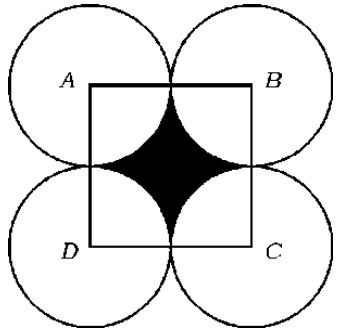Solution:

Given,

Side of the square ABCD = 14 cm

Area of square ABCD = (14)2 = 196 cm2

Radius of each quadrant of the circle = r = 14/2 = 7 cm

Area of one quadrant of circle = (1/4) × πr2

= (1/4) × (22/7) × (7)2

= (1/4) × 22 × 7

= 154/4 cm2

Area of 4 quadrants = 4 (154/4) = 154 cm2

Area of the shaded region = Area of square ABCD – Area of 4 quadrants with centres A, B, C and D

= 196 – 154

= 42 cm2

20. Draw a circle of radius 4 cm and construct a pair of tangents such that the angle between them is 60°.

Solution:

Steps of construction:

(i) Draw a circle with centre O and radius 4 cm.

(ii) Take a point Q on the circumference of the circle and join OQ.

(iii) Draw a perpendicular to QP at point Q.

(iv) Draw a radius OR, making an angle of 120° (180° − 60°) with OQ.

(v) Draw a perpendicular to RP at point R, which intersects the previous perpendicular at P.

Therefore, PQ and PR are the required tangents inclined at an angle of 60°.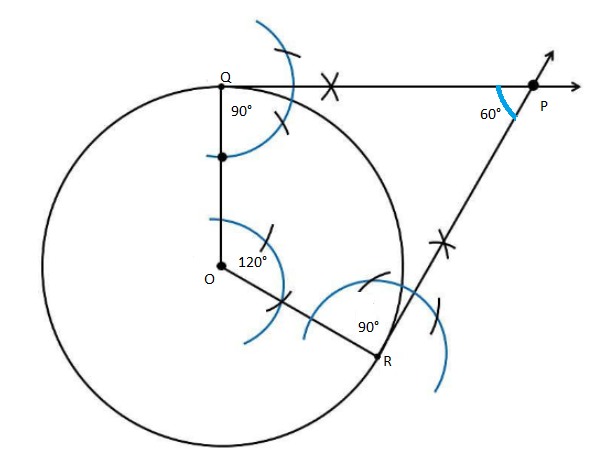21. Find the coordinates of the point which divides the line segment joining the points A(4, -3) and B(8, 5) in the ratio 3 : 1 internally.

Solution:

Let P(x, y) be the point which intersects the line segment joining the points A(4, -3) and B(8, 5) internally in the ratio 3 : 1.

Here,

(x1, y1) = (4 ,-3)

(x2, y2) = (8, 5)

m1 : m2 = 3 : 1

Using the section formula,

x – coordinate:

x = (m1x2 + m2x1)/(m1 + m2)

= [3(8) + 1(4)]/ (3 + 1)

= (24 + 4)/4

= 28/4

= 7

y – coordinate

y = (m1y2 + m2y1)/(m1 + m2)

= [3(5) + 1(-3)]/ (3 + 1)

= (15 – 3)/4

= 12/4

= 3

Hence, the coordinates of the required points are (7, 3).

22. Prove that 3 + √5 is an irrational number.

Solution:

Let 3 + √5 be a rational number.

Thus, 3 + √5 = a/b, where a, b are coprime integers and b ≠ 0.

⇒ √5 = (a/b) – 3

⇒ √5 = (a – 3b)/b

Since a and b are integers, (a – 3b)/b is a rational number.

⇒ √5 is also a rational number.

This is the contradiction to the fact that √5 is an irrational number.

Hence, our assumption that 3 + √5 is a rational number is wrong.

Therefore, 3 + √5 is an irrational number.

Hence proved.

23. The sum and product of the zeroes of a quadratic polynomial p(x) = ax2 + bx + c are -3 and 2 respectively. Show that b + c = 5a.

Solution:

p(x) = ax2 + bx + c

Sum of the zeroes = -coefficient of x/coefficient of x2

-3 = -b/a

⇒ b/a = 3

⇒ b = 3a ….(i)

Product of the zeroes = constant term/coefficient of x2

2 = c/a

⇒ c/a = 2

⇒ c = 2a ….(ii)

b + c = 3a + 2a

b + c = 5a

24. Find the quotient and the remainder when p(x) = 3x3 + x2 + 2x + 5 is divided by g(x) = x2 + 2x + 1.

Solution:

Given,

p(x) = 3x3 + x2 + 2x + 5

g(x) = x2 + 2x + 1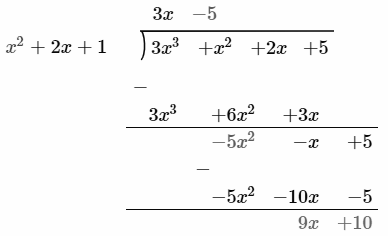Therefore,

Quotient = q(x) = 3x – 5

Remainder = r(x) = 9x + 10

25. Solve 2x2 – 5x + 3 = 0 by using formula.

Solution:

Given quadratic equation is 2x2 – 5x + 3 = 0

Comparing with the standard form ax2 + bx + c = 0,

a = 2, b = -5 and c = 3

Discriminant = D

= b2 – 4ac

= (-5)2 – 4(2)(3)

= 25 – 24

= 1

x = [-b ± √D]/2a

= [-(-5) ± √1]/ 2(2)

= (5 ± 1)/4

x = (5 + 1)/4, x = (5 – 1)/4

x = 6/4, x = 4/4

x = 3/2, x = 1

26. The length of a rectangular field is 3 times its breadth. If the area of the field is 147 sq.m, find its length and breadth.

Solution:

Let x be the breadth and 3x be the length of the rectangular field.

According to the given,

x(3x) = 147 sq.m

3x2 = 147

x2 = 147/3

x2 = 49

x = √49

x = 7 m

Therefore, breadth = x = 7 m

Length = 3x = 3(7) = 21 m

27. If sin θ = 12/13, find the values of cos θ and tan θ.

Solution:

Given,

sin θ = 12/13

sin θ = Opposite/Hypotenuse = AB/AC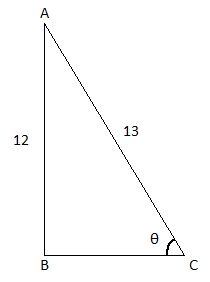In right triangle ABC,

By Pythagoras theorem,

AC2 = AB2 + BC2

BC2 = AC2 – AB2

= (13)2 – (12)2

= 169 – 144

= 25

BC = √25 = 5

cos θ = BC/AC = 5/13

tan θ = AB/BC = 12/5

OR

If √3 tan θ = 1 and θ is acute, find the value of sin 3θ + cos 2θ.

Solution:

Given,

√3 tan θ = 1 and θ is acute

tan θ = 1/√3

tan θ = tan 30°

θ = 30°

Now,

sin 3θ + cos 2θ = sin 3(30°) + cos 2(30°)

= sin 90° + cos 60°

= 1 + (1/2)

= 3/2

28. Prove that (1 + cos θ)/(1 – cos θ) = (cosec θ + cot θ)2.

Solution:

LHS = (1 + cos θ)/(1 – cos θ)

= [(1 + cos θ)/(1 – cos θ)] × [(1 + cos θ)/(1 + cos θ)]

= (1 + cos θ)2/ (1 – cos2θ)

Using the identity sin2A + cos2A = 1,

= [1 + cos2θ + 2 cos θ]/ sin2θ

= (1/sin2θ) + (cos2θ/ sin2θ) + (2 cos θ/ sin2θ)

= cosec2θ + cot2θ + 2 × (1/sin θ) × (cos θ/sin θ)

= cosec2θ + cot2θ + 2 cosec θ cot θ

= (cosec θ + cot θ)2

= RHS

Hence proved.

29. A cubical die numbered from 1 to 6 is rolled twice. Find the probability of getting the sum of numbers on its faces is 10.

Solution:

Total number of possible outcomes = 62 = 36

i.e. n(S) = 36

Let E be the event that the sum of numbers on dices is 10.

E = {(4, 6), (6, 4), (5, 5)}

Number of outcomes favourable to E = n(E) = 3

P(E) = n(E)/n(S)

= 3/36

= 1/12

Therefore, the required probability is 1/12.

30. The radii of two circular ends of a frustum of a cone shaped dustbin are 15 cm and 8 cm. If its depth is 63 cm, find the volume of the dustbin.

Solution:

Given,

The radii of two circular ends of a frustum of a cone shaped dustbin are 15 cm and 8 cm.

R = 15 cm

r = 8 cm

Depth = h = 63 cm

Volume = (πh)/3 (R2 + r2 + Rr)

= (1/3) × (22/7) × 63 × [(15)2 + (8)2 + 15 × 8]

= (22 × 3) × (225 + 64 + 120)

= 66 × 409

= 26994 cm3

Therefore, the volume of the dustbin is 26994 cm3.

SECTION – IV

31. Prove that “the lengths of tangents drawn from an external point to a circle are equal”.

Solution:

Let O be the centre of the circle.

PQ and PR be the two tangents drawn to the circle from an external point P.

Join OP, OQ and OR.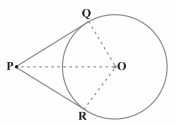We know that radius is perpendicular to the tangent through the point of contact.

Thus, ∠OQP = ∠ORP = 90°

In right ΔOQP and ΔORP,

OQ = OR (radius of the same circle)

OP = OP (common)

By RHS congruence criterion,

ΔOQP ≅ ∆ORP

By CPCT,

PQ = PR

Hence proved.

OR

In the given figure, PQ and RS are two parallel tangents to a circle with centre O and another tangent AB with point of contact C intersecting PQ at A and RS at B. Prove that ∠AOB = 90°.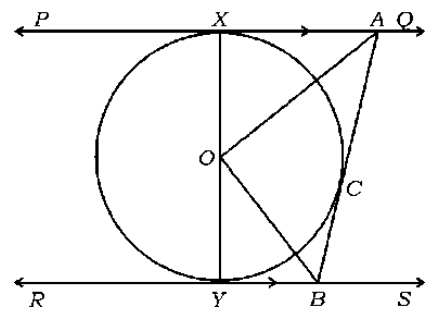Solution:

Given,

PQ and RS are two parallel tangents to a circle with centre O.

Join OC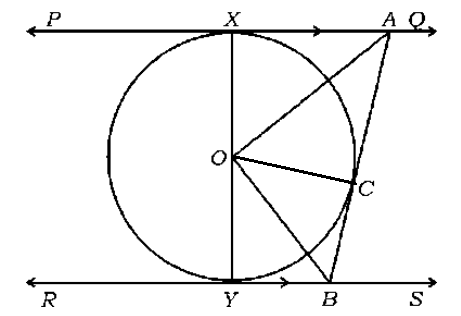In ΔOXA and ΔOCA,

OX = OC (radius of the same circle)

AO = AO (common)

AX = AC (tangents drawn from point A)

By SSS congruence criterion,

△OXA ≅ △OCA

Similarly,

△OYB ≅ △OCB

Thus,

∠XOA = ∠COA ….(i)

∠YOB = ∠COB ….(ii)

From the given figure, XOY is a straight line and is the diameter of the circle.

∠XOA + ∠COA + ∠COB + ∠YOB = 180°

Substituting (i) and (ii) in the above equation,

2∠COA + 2∠COB = 180°

⇒ ∠ =COA + ∠COB = 90°

∴ ∠ AOB = 90°

Hence proved.

32. Calculate the median of the following frequency distribution table:

 Class-interval Frequency (fi) 1 – 4 6 4 – 7 30 7 – 10 40 10 – 13 16 13 – 16 4 16 – 19 4 ∑fi = 100

Solution:

Cumulative frequency distribution table

 Class-interval Frequency (fi) Cumulative frequency 1 – 4 6 6 4 – 7 30 36 = cf l = 7 – 10 40 = f 76 10 – 13 16 92 13 – 16 4 96 16 – 19 4 100

N/2 = 100/2 = 50

Cumulative frequency greater or equal to N/2 is 76, which lies in the class interval 7 – 10

Median class = 7 – 10

Lower limit of the median class = l = 7

Frequency of the median class = f = 40

Cumulative frequency above the median class = cf = 36

Class height = h = 3

Median = l + [(N/2 – cf)/f] × h

= 7 + [(50 – 36)/40] × 3

= 7 + (14 × 3)/40

= 7 + (42/40)

= 7 + 1.05

= 8.05

OR

Calculate the mode for the following frequency distribution table.

 Class-interval Frequency (fi) 10 – 25 2 25 – 40 3 40 – 55 7 55 – 70 6 70 – 85 6 85 – 100 6 ∑fi = 30

Highest frequency = 7

Thus, modal class is 40 – 55

Frequency of the modal class = fm = 7

Lower limit of the modal class = l = 40

Frequency of the class preceding the modal class = f1 = 3

Frequency of the class succeeding the modal class = f2 = 6

Class height = h = 15

Mode = l + [(fm – f1)/ (2fm – f1 – f2)] × h

= 40 + [(7 – 3)/ (2 × 7 – 3 – 6)] × 15

= 40 + [4/ (14 – 9)] × 15

= 40 + (4 × 15)/5

= 40 + 12

= 52

33. During the medical check-up of 35 students of a class, their weights were recorded as follows. Draw a less than type of ogive for the given data:

 Weight (in kg) Number of students Less than 38 0 Less than 40 3 Less than 42 5 Less than 44 9 Less than 46 14 Less than 48 28 Less than 50 32 Less than 52 35

Solution:

From the given data, to draw less than ogive, choose the upper limits of the class intervals on the x-axis and cumulative frequencies on the y-axis by choosing the convenient scale.

Plot the points corresponding to the ordered pairs given by:

(38, 0), (40, 3), (42, 5), (44, 9),(46, 14), (48, 28), (50, 32) and (52, 35) on a graph paper.

Join all these points to get a smooth curve.

The curve obtained in the graph is known as less than type ogive.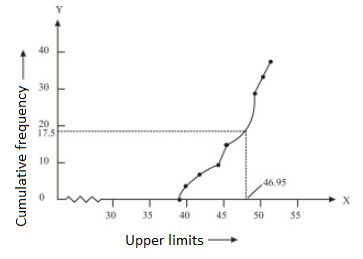34. The seventh term of an Arithmetic progression is four times its second

term and twelfth term is 2 more than three times of its fourth term. Find

the progression.

Solution:

Let a be the first term and d be the common difference of an AP.

nth term of an AP is an = a + (n – 1)d

According to the given,

a7 = 4a2 ….(i)

and a12 = 3a4 + 2 ….(ii)

From (i),

a + 6d = 4(a + d)

a + 6d = 4a + 4d

⇒ 4a – a = 6d – 4d

⇒ 3a = 2d ….(iii)

From (ii),

a + 11d = 3(a + 3d) + 2

a + 11d = 3a + 9d + 2

⇒ 3a – a = 11d – 9d – 2

⇒ 2a = 2d – 2

⇒ 2a = 3a – 2

⇒ 3a – 2a = 2

⇒ a = 2

Substituting a = 2 in (iii),

3(2) = 2d

⇒ d = 6/2

⇒ d = 3

Hence, the required AP is 2, 5, 8, 11,….

OR

A line segment is divided into four parts forming an Arithmetic progression. The sum of the lengths of 3rd and 4th parts is three times the sum of the lengths of the first two parts. If the length of the fourth part is 14 cm, find the total length of the line segment.

Solution:

Let a1, a2, a3 and a4 be the lengths of four parts which form an AP.

According to the given,

a3 + a4 = 3(a1 + a2)….(i)

a4 = 14 cm ….(ii)

From (i),

a + 2d + a + 3d = 3(a + a + d)

2a + 5d = 3(2a + d)

2a + 5d = 6a + 3d

⇒ 6a – 2a = 5d – 3d

⇒ 4a = 2d

⇒ 2a = d ….(iii)

From (ii),

a + 3d = 14

Substituting (iii),

a + 3(2a) = 14

a + 6a = 14

7a = 14

a = 14/7 = 2

Substituting a = 2 in (iii),

d = 2(2) = 4

Now,

a1 = a = 2 cm

a2 = a + d = 2 + 4 = 6 cm

a3 = a2 + d = 6 + 4 = 10 cm

Therefore, the total length of the line segment = (2 + 6 + 10 + 14) cm = 32 cm

35. The vertices of a Δ ABC are A(-3, 2), B(-1, -4) and C (5, 2). If M and N are the mid-points of AB and AC respectively, show that 2 MN = BC.

Solution:

Given,

Vertices of a triangle ABC are A(-3, 2), B(-1, -4) and C(5, 2).

M is the midpoint of AB.

Using midpoint formula,

M = [(-3 – 1)/2, (2 – 4)/2]

= (-4/2, -2/2)

= (-2, -1)

N is the midpoint of AC.

N = [(-3 + 5)/2, (2 + 2)/2]

= (2/2, 4/2)

= (1, 2)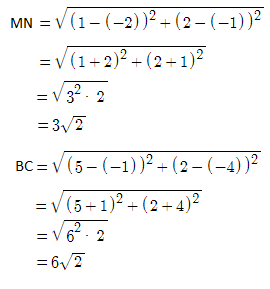Therefore, 2 MN = BC

OR

The vertices of a Δ ABC are A(-5, -1), B(3, -5), C(5, 2). Show that the area of the Δ ABC is four times the area of the triangle formed by joining the mid-points of the sides of the triangle ABC.

Solution:

Given,

Vertices of a triangle ABC are A(-5, -1), B(3, -5), C(5, 2).

Let D, E and F be the midpoints of AB, BC and AC of triangle ABC.

Using the midpoint formula,

D = [(-5 + 3)/2, (-1 – 5)/2]

= (-2/2, -6/2)

= (-1, -3)

E = [(3 + 5)/2, (-5 + 2)/2]

= (8/2, -3/2)

= (4, -3/2)

F = [(-5 + 5)/2, (-1 + 2)/2]

= (0/2, ½)

= (0, ½)

Area of triangle ABC = ½[x1(y2 – y3) + x2(y3 – y1) + x3(y1 – y2)]

= ½[-5(-5 – 2) + 3(2 + 1) + 5(-1 + 5)]

= ½ [-5(-7) + 3(3) + 5(4)]

= ½ (35 + 9 + 20)

= ½ (64)

= 32 sq.units

Area of triangle DEF = ½ [-1(-3/2 – ½) + 4(½ + 3) + 0(-3 + 3/2)]

= ½ [-1(-2) + 4(7/2) + 0]

= ½ [2 + 14]

= ½ (16)

= 8

Therefore, Area of triangle ABC = 4 × Area of triangle DEF

36. Construct a triangle with sides 5 cm, 6 cm and 7 cm and then construct another triangle whose sides are 7/5 of the corresponding sides of the first triangle.

Solution:

Steps of construction:

(i) Draw a line segment AB of length 5 cm.

(ii) Taking A and B as centres, draw the arcs of radius 6 cm and 5 cm respectively, which intersect each other at C.

(iii) Join AC and BC and ΔABC is obtained.

(iv) Draw a ray AX which makes an acute angle with AB on the opposite side of vertex C.

(v) Locate the 7 points on AX such as AA1 = A1A2 = A2A3 = A3A4 = A4A5 = A5A6 = A6A7.

(vi) Join the points BA5 and draw a line from A7 to BA5 which is parallel to the line BA5 this wil intersect the produced AB at B’.

(vii) Draw a line from B’ parallel to AC so that it intersects the produced AC at C’.

Therefore, ΔAB’C’ is the required triangle.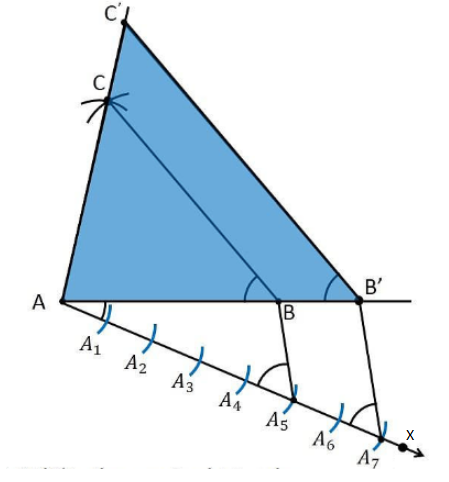SECTION – V

37. Find the solution of the following pairs of linear equation by the graphical method:

2x + y = 6

2x – y = 2

Solution:

Given pair of equations is:

2x + y = 6

2x – y = 2

Consider the first equation:

2x + y = 6

y = -2x + 6

Table for the respective x and y values is:

 x 0 1 2 3 y 6 4 2 0

Now, consider the second equation:

2x – y = 2

y = 2x – 2

Table for the respective x and y values is:

 x 0 1 2 3 y -2 0 2 4

Graph: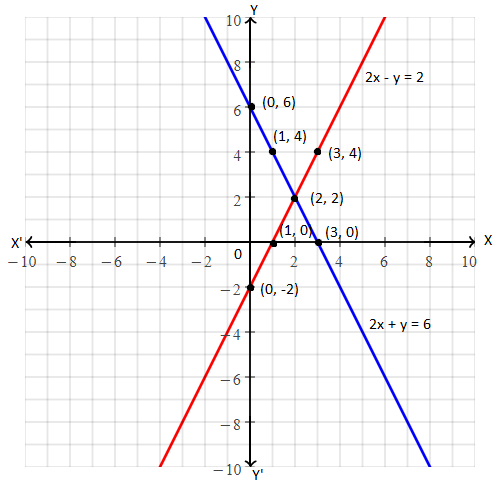The lines representing the given pair of equations intersecting each other at (2, 2)

Therefore x = 2 and y = 2 is the required solution.

38. The angles of elevation of the top of a tower from two points at a distance of 4 m and 9 m from the base of the tower and in the same straight line with it are complementary. Find the height of the tower.

Solution:

Let AB be the tower and C, D be the points of observation.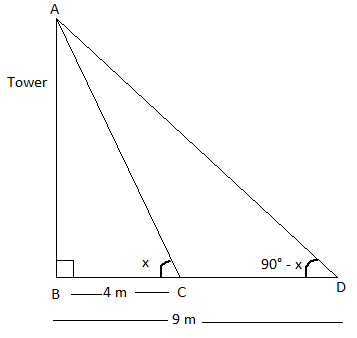In right ΔABC,

tan x = AB/BC

tan x = AB/4

AB = 4 tan x ….(i)

In right ΔABD,

tan (90°- x) = AB/BD

cot x = AB/9

AB = 9 cot x ….(ii)

Multiplying (i) and (ii),

AB2 = 9 cot x × 4 tan x

⇒ AB2 = 36 cot x = 1/tan x)

⇒ AB = ± 6

Height cannot be negative.

Therefore, the height of the tower is 6 m.

39. The bottom of a right cylindrical shaped vessel made from metallic sheet is closed by a cone shaped vessel as shown in the figure. The radius of the circular base of the cylinder and radius of the circular base of the cone are each equal to 7 cm. If the height of the cylinder is 20 cm and height of cone is 3 cm, calculate the cost of milk to fill completely this vessel at the rate of Rs. 20 per litre.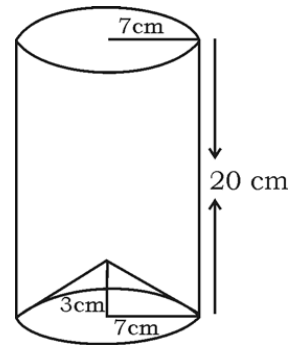Solution:

Given,

Height of the cylinder = H = 20 cm

Height of the cone = h = 3 cm

Radius of cylinder & cone = r = 7 cm

Volume of the vessel = Volume of cylinder – Volume of cone

= πr2H – (1/3)πr2h

= (22/7) × 7 × 7 × 20 – (1/3) × (22/7) × 7 × 7 × 3

= (22/7) × 7 × 7 [20 – 1]

= 154 × 19

= 2926 cm3

= 2926/1000 lit

= 2.926 lit

Cost of 1 litre milk = Rs. 20

Total cost of milk which will fill the vessel = Rs. 20 × 2.926

= Rs. 58.52

OR

A hemispherical vessel of radius 14 cm is fully filled with sand. This sand is poured on a level ground. The heap of sand forms a cone shape of height 7 cm. Calculate the area of ground occupied by the circular base of the heap of the sand.

Solution:

Given,

Radius of the hemispherical vessel = R = 14 cm

Height of the cone = h = 7 cm

Let r be the radius of conical heap.

Volume of hemispherical vessel = Volume of conical heap

(2/3)πr3 = (1/3)πr2h

2 × 14 × 14 × 14 = r2 × 7

r2 = 2 × 2 × 14 × 14

r2 = (2)2 × (14)2

r = 2 × 14

r = 28 cm

Area of the circular base of conical heap = πr2

= (22/7) × 28 × 28

= 22 × 4 × 28

= 2464 cm2

40. Prove that “the ratio of the areas of two similar triangles is equal to the square of the ratio of their corresponding sides”.

Solution:

Given,

Two triangles ABC and PQR such that ∆ABC ~ ∆PQR

Draw altitudes AM and PN of two triangles ABC and PQR respectively.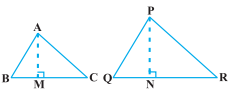ar(ΔABC) = 1/2 BC × AM

ar(ΔPQR) = 1/2 QR × PN

Now,

ar(ΔABC)/ar(ΔPQR) = [(1/2) BC × AM] / [(1/2) QR × PN]

= (BC × AM)/ (QR × PN) ….(i)

In ΔABM and ΔPQN,

∠B = ∠Q (∆ABC ~ ∆PQR)

∠M = ∠N (each 90°)

Thus, ∆ABM ~ ∆PQN (by AA similarity criterion)

⇒ AM/PN = AB/PQ ….(ii)

∆ABC ~ ∆PQR (given)

AB/PQ = BC/QR = CA/RP ….(iii)

From (i) and (iii),

ar(ΔABC)/ar(ΔPQR) = (AB/PQ) × (AM/PN)

= (AB/PQ) × (AB/PQ) [From (ii)]

= (AB/PQ)2

Similarly,

ar(ΔABC)/ar(ΔPQR) = (AB/PQ)2 = (BC/QR)2 = (CA/RP)2

Hence proved.